# Selina Solutions Concise Physics Class 10 Chapter 11 Calorimetry

Selina Solutions Concise Physics Class 10 Chapter 11 Calorimeter provides information pertaining to the properties of heat and its measurement using a calorimeter. Subject experts have designed the solutions in order to boost students’ exam preparation. Students are advised to practise these Selina Solutions Concise Physics Class 10, which help them to solve the questions with ease and precision. Selina Solutions provide very accurate answers, which also improves the understanding capacity among them.

Selina Solutions Concise Physics Class 10 are crafted in an interactive manner to create interest among students during study hours. These solutions are the best study materials, as they contain answers which are vital from an exam point of view. Selina Solutions are helpful to students who want to excel with good marks in the examination. Download the Selina Solutions Concise Physics Class 10 PDF with the help of links provided here.

## Selina Solutions Concise Physics Chapter 11 Calorimetry:-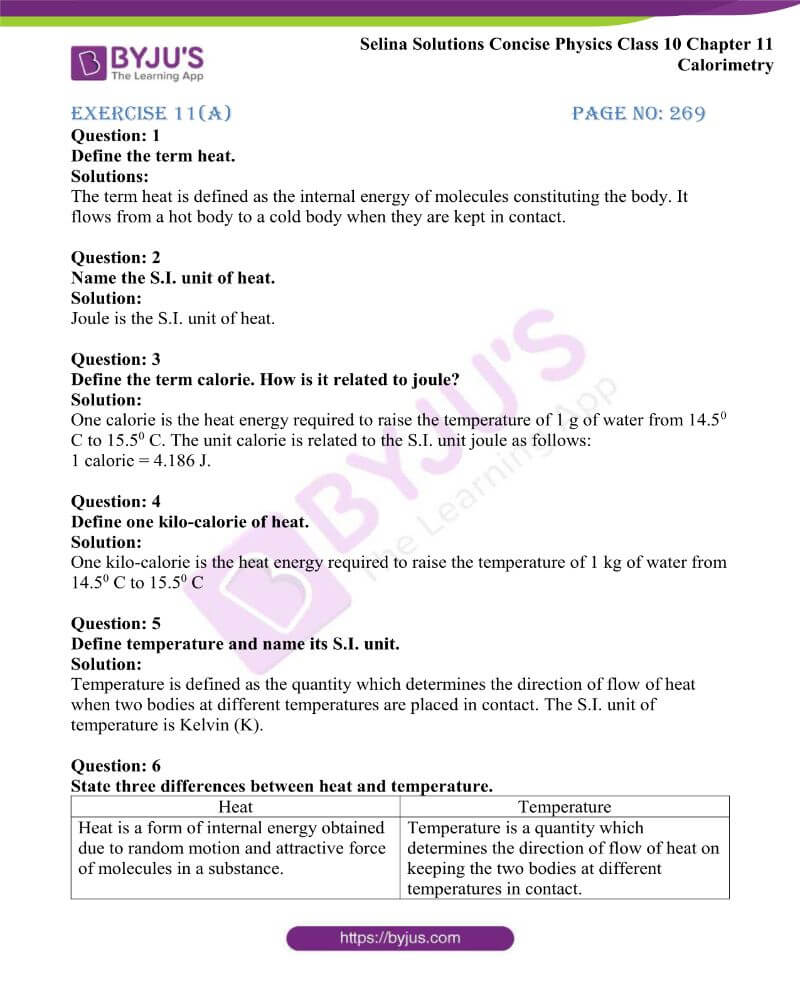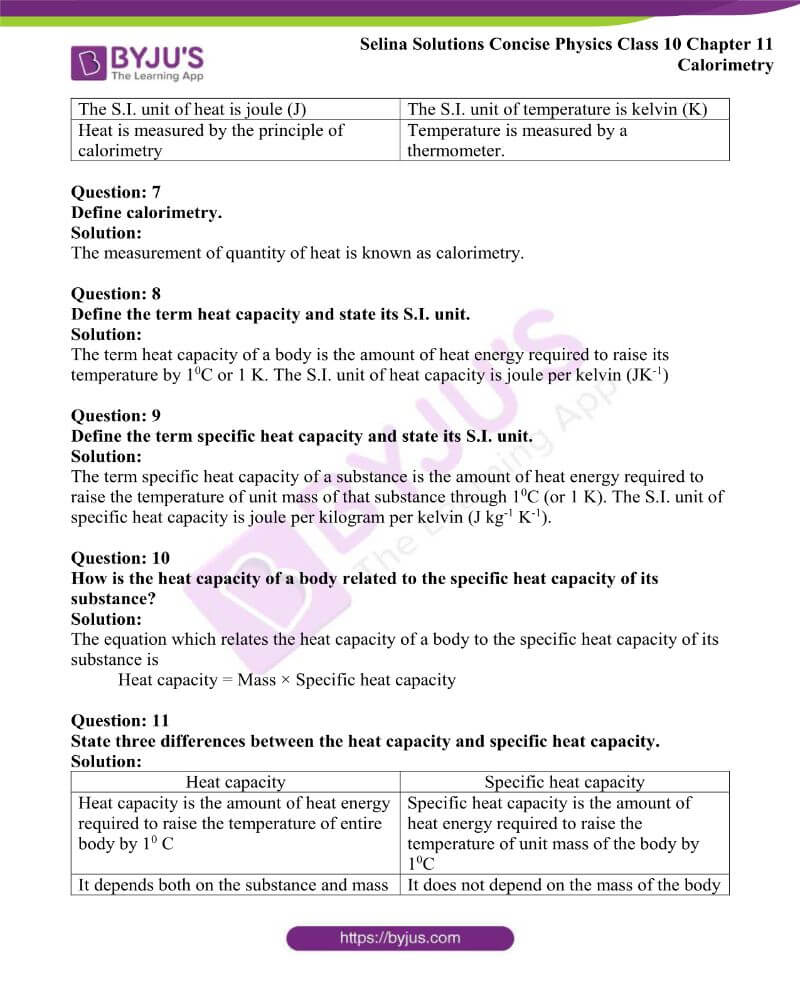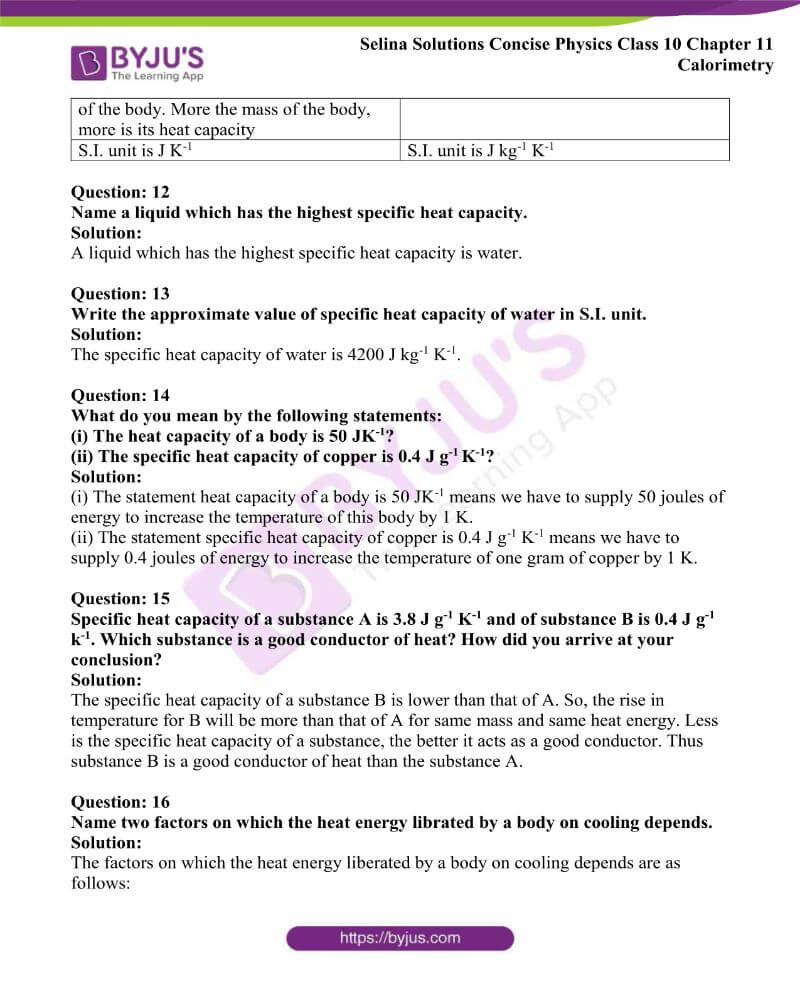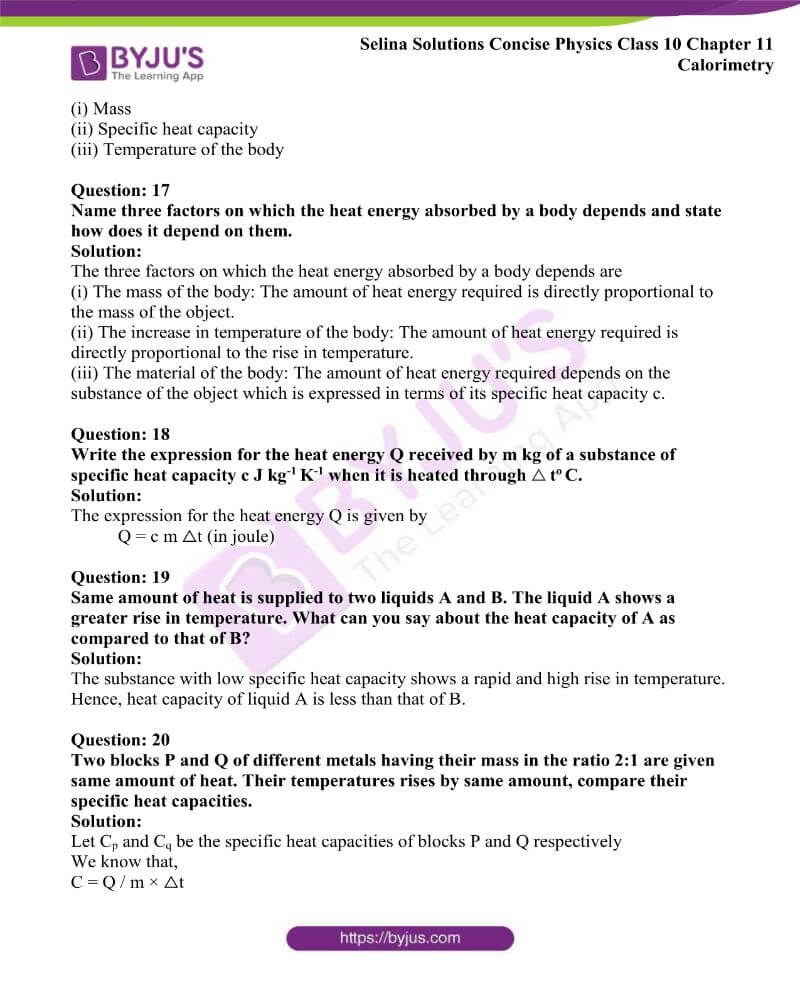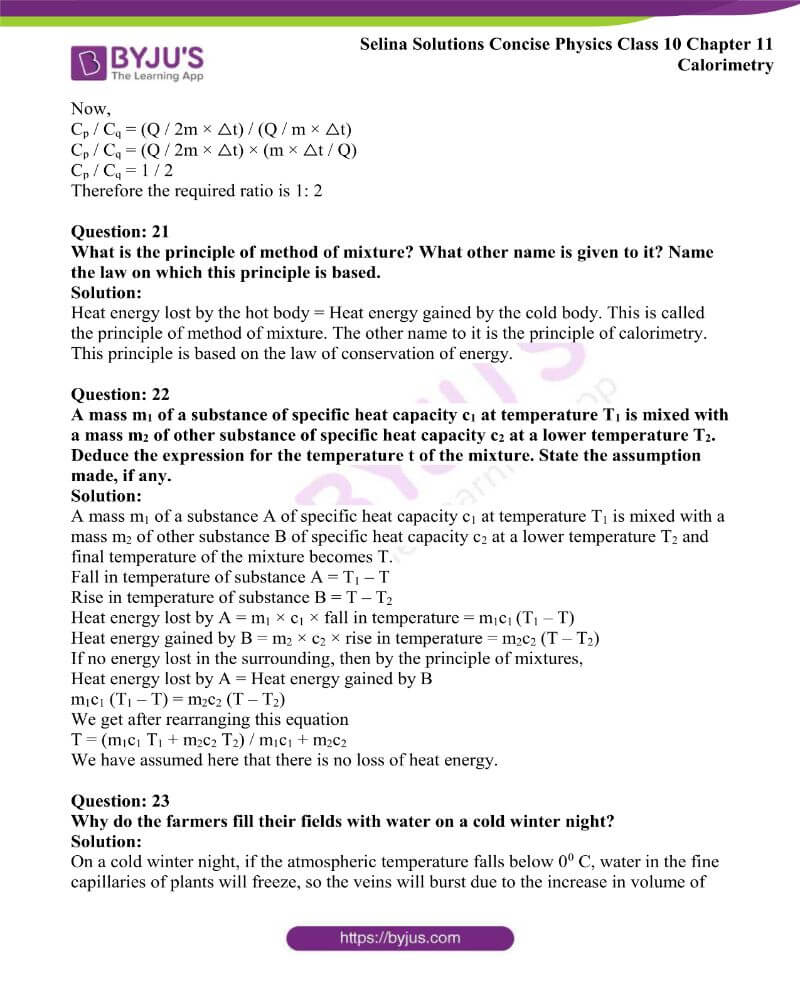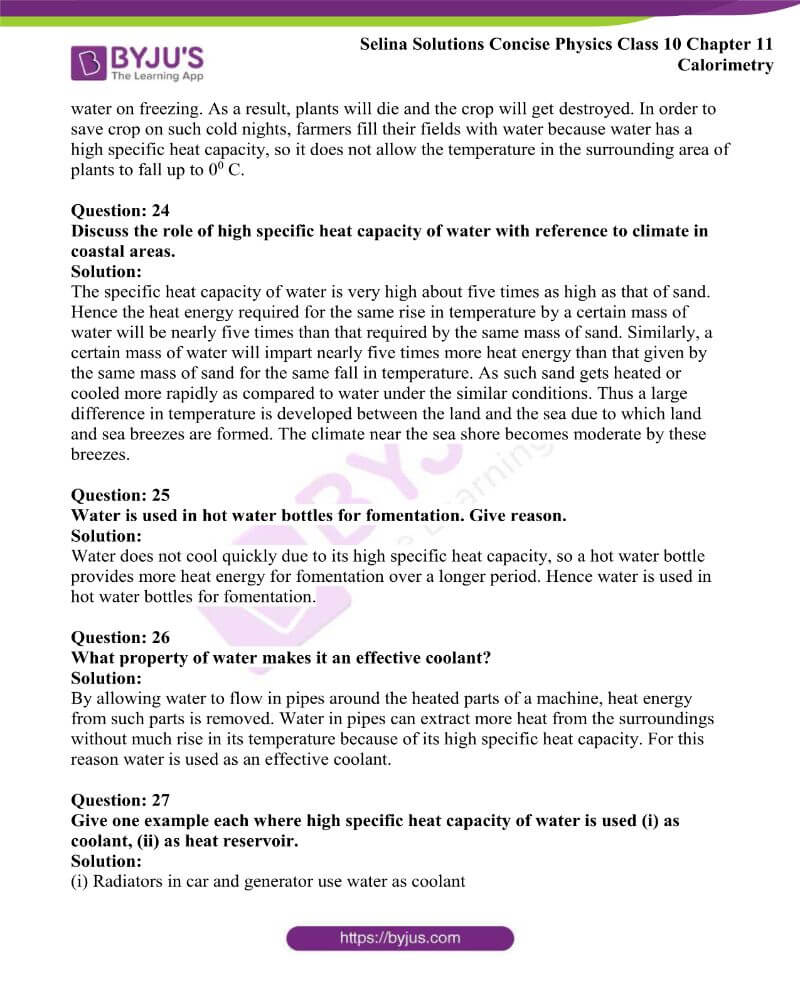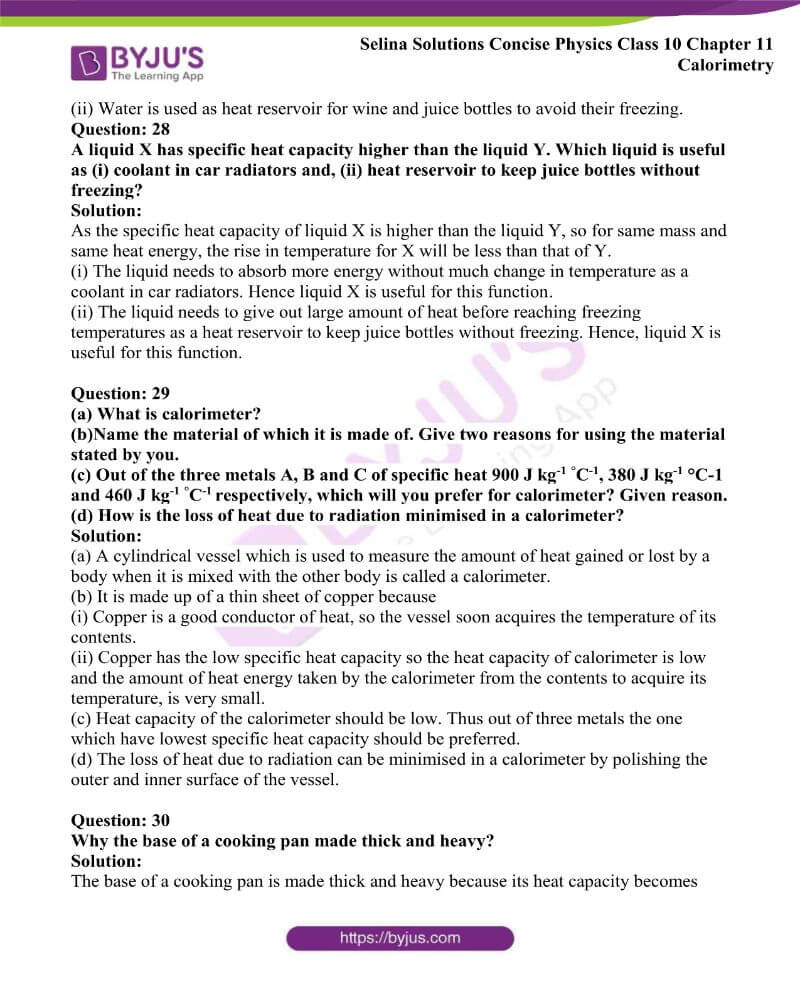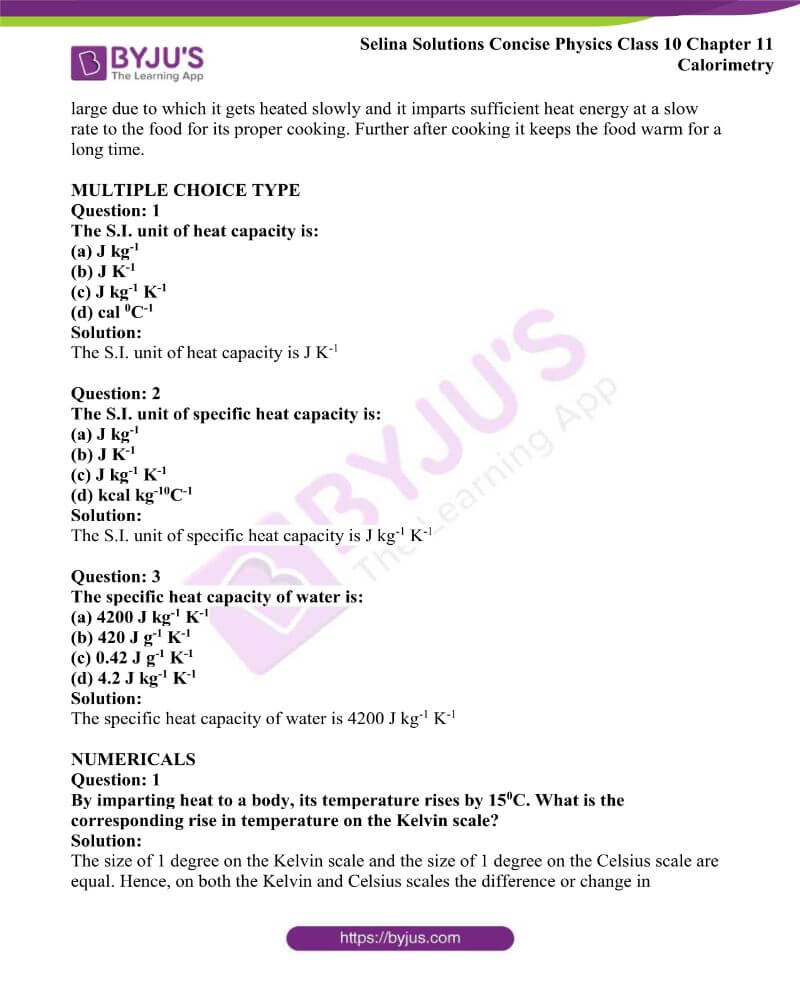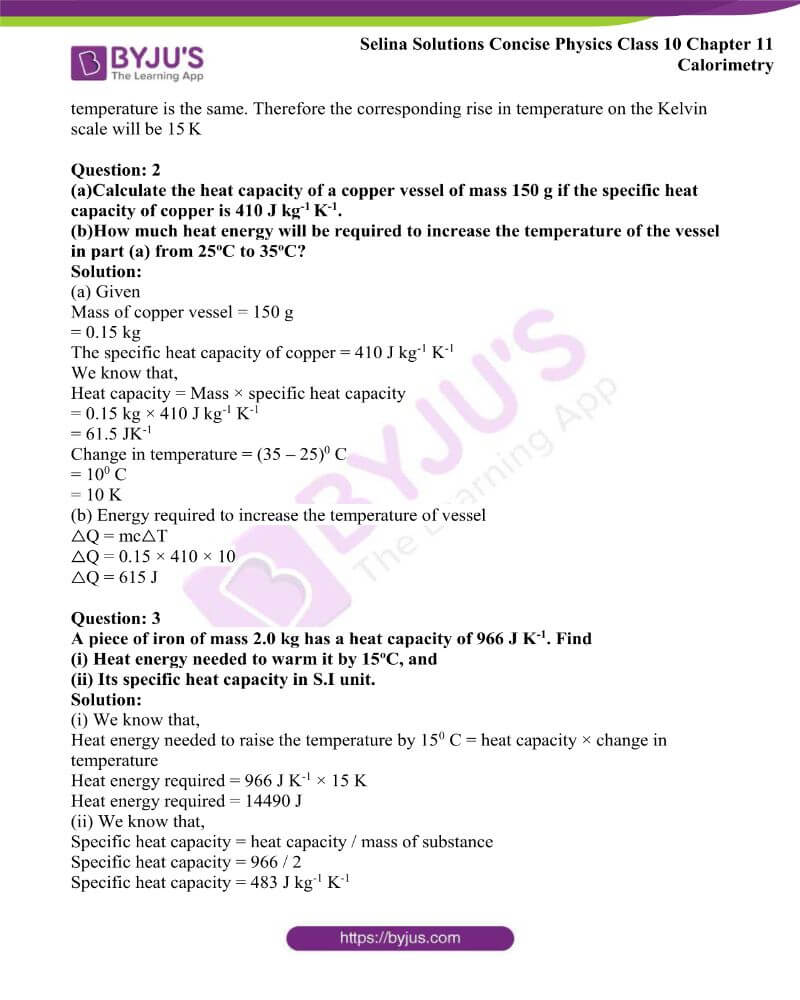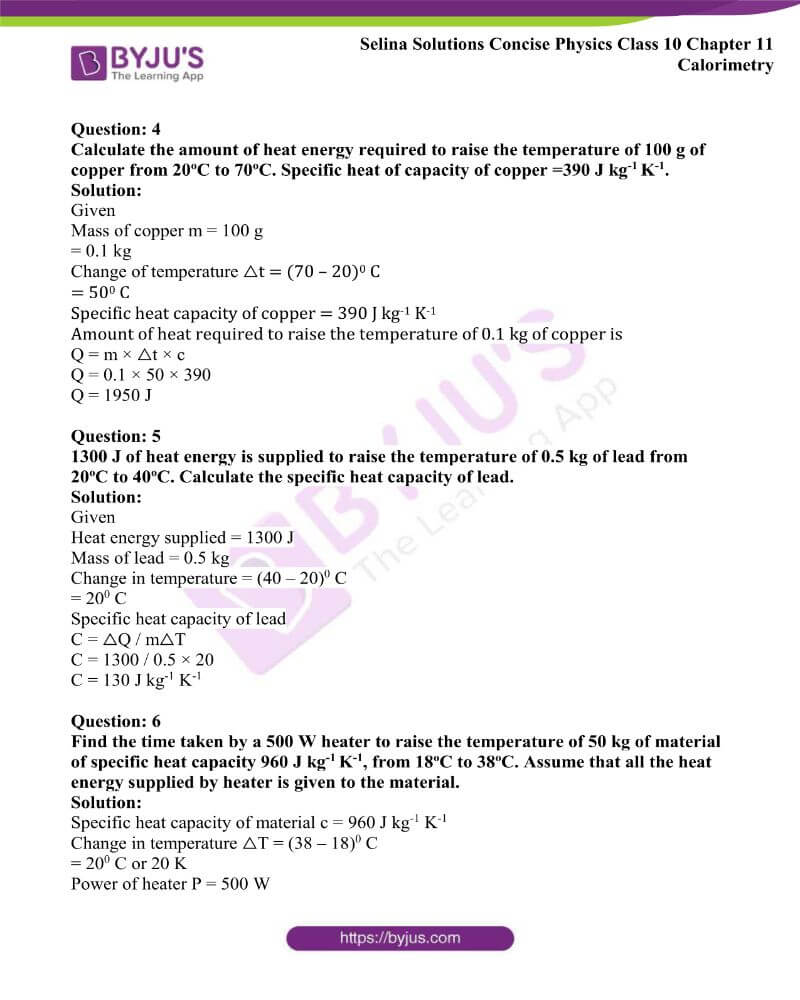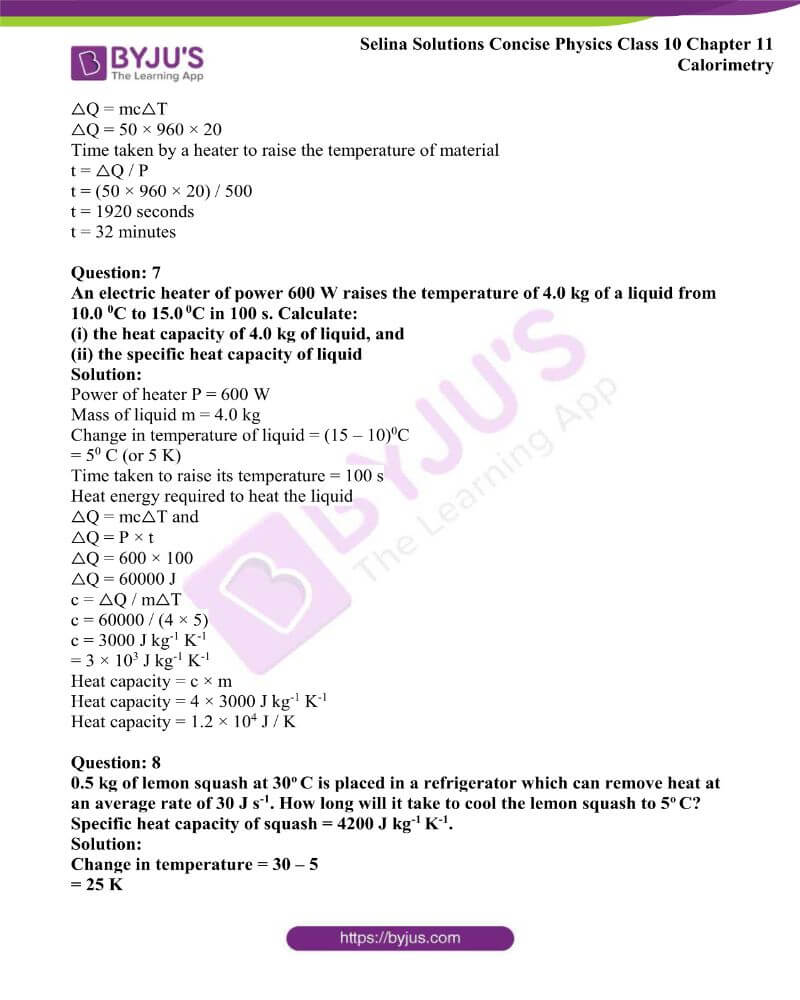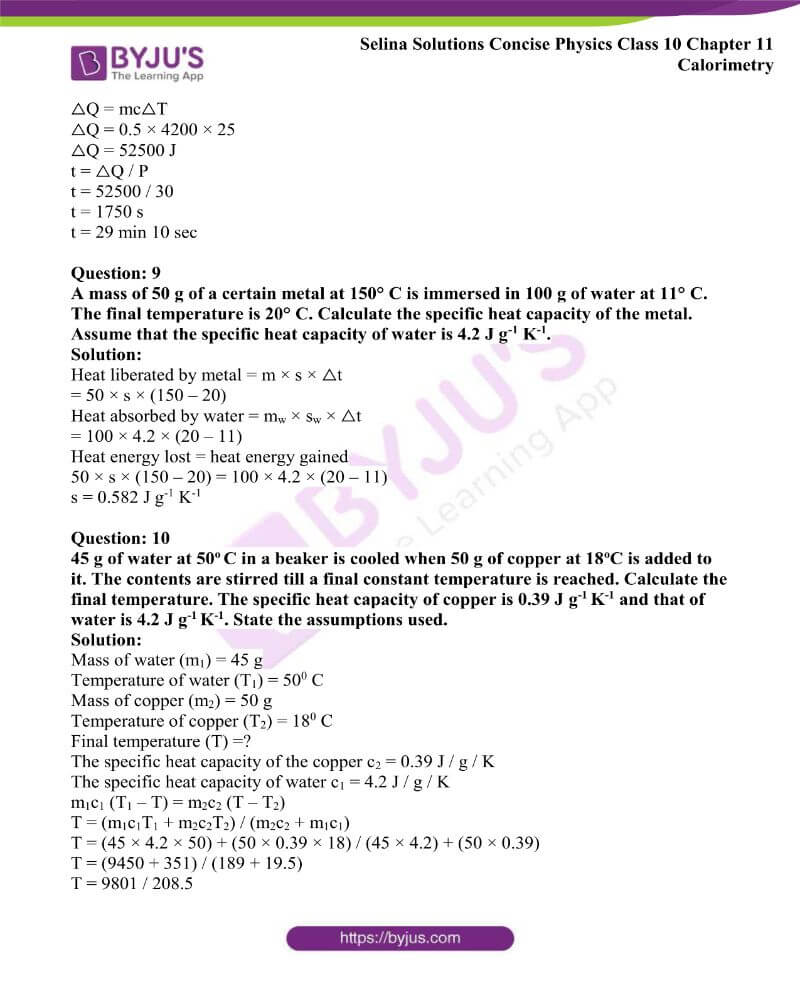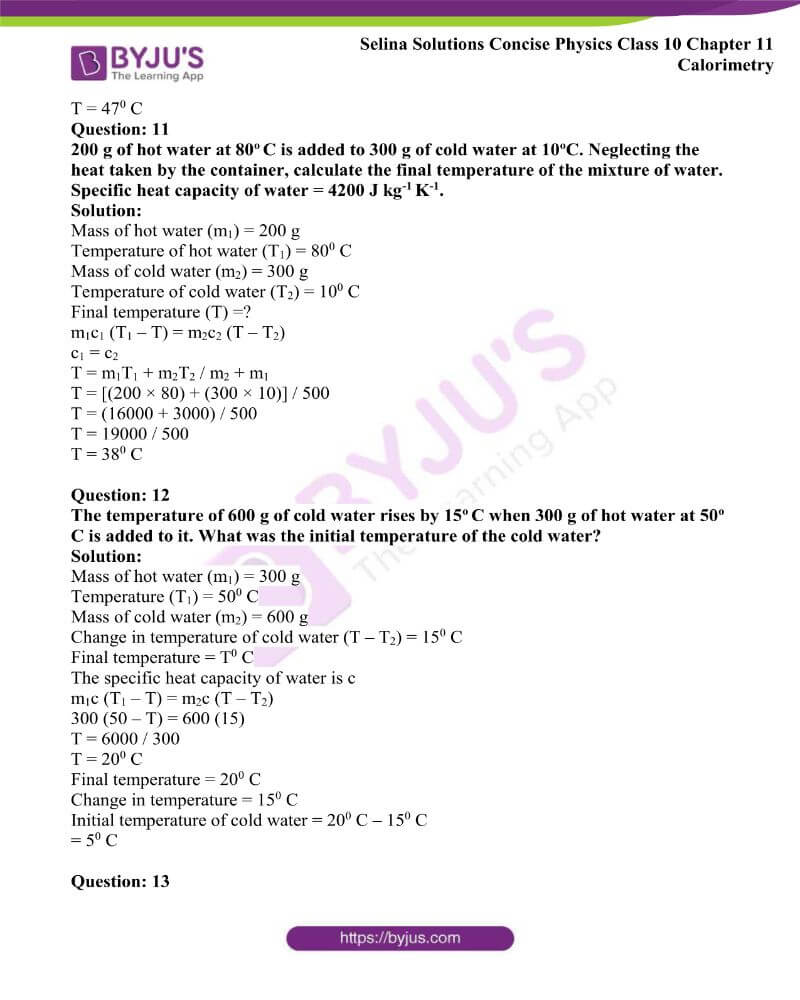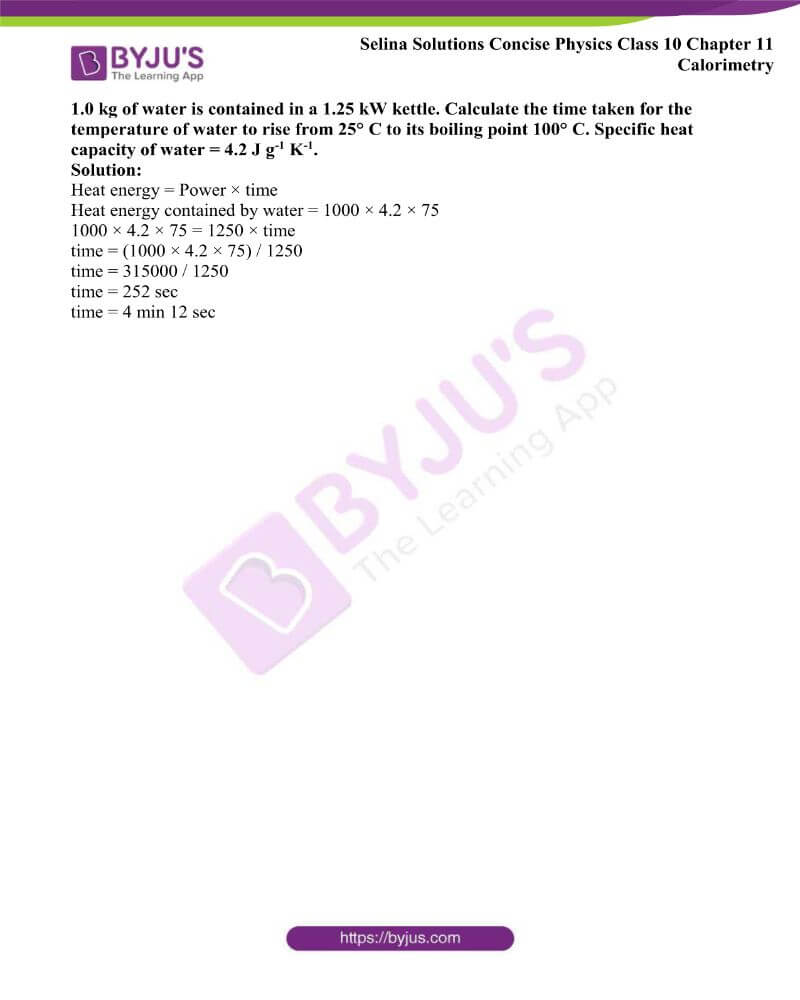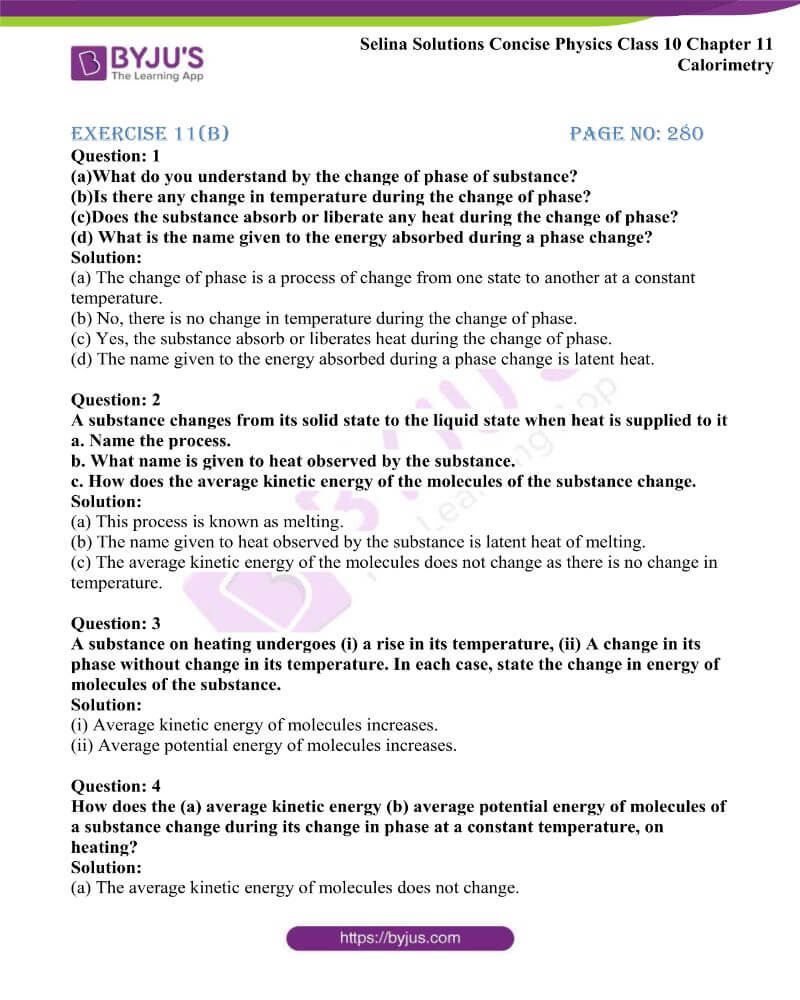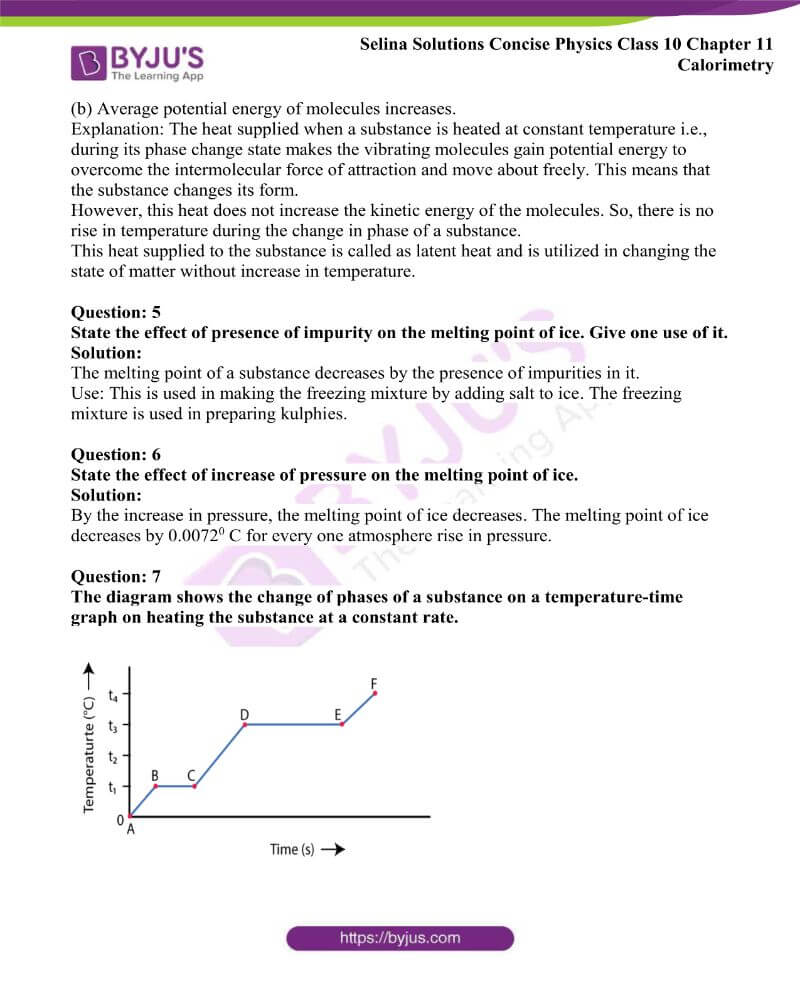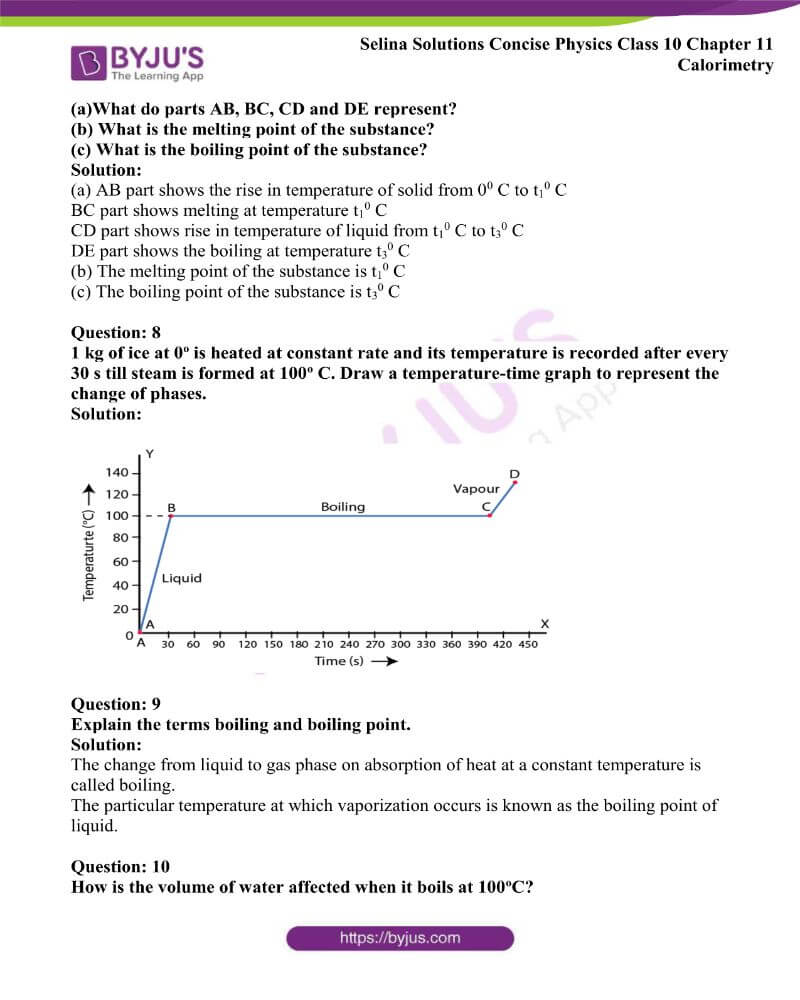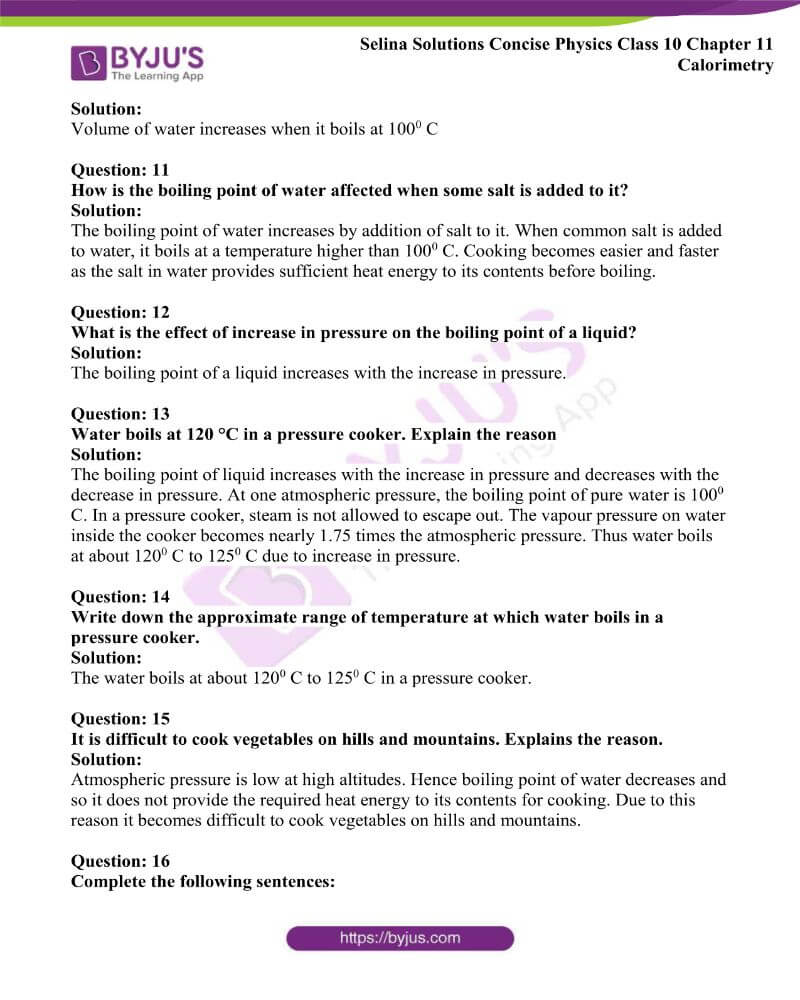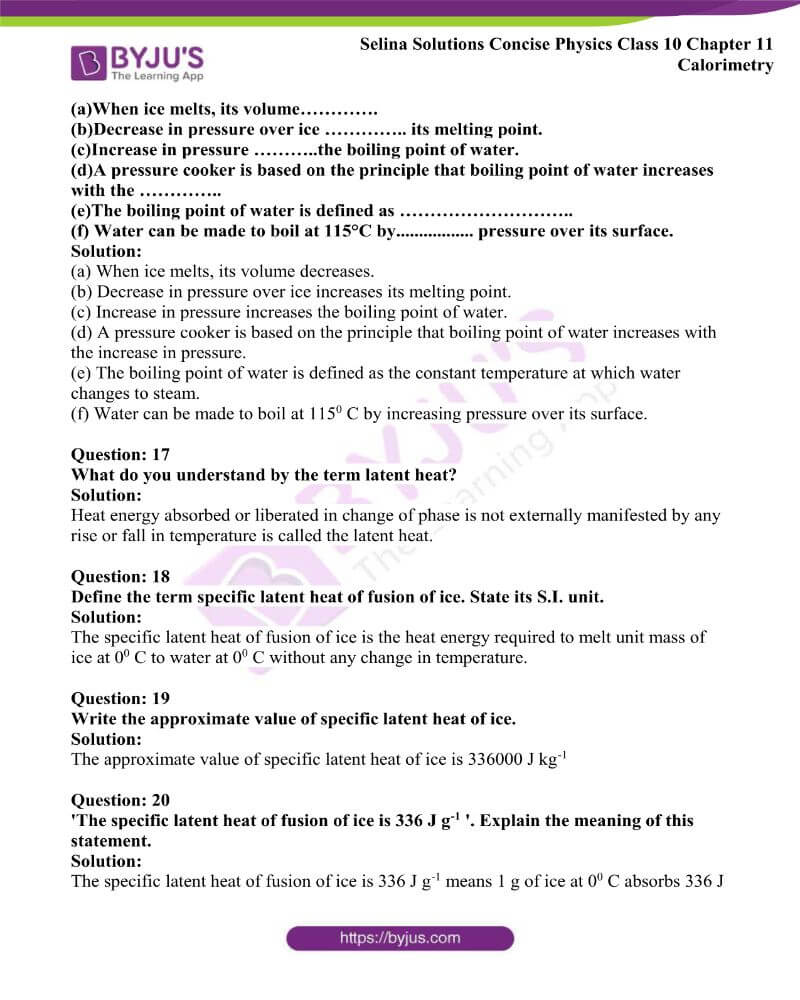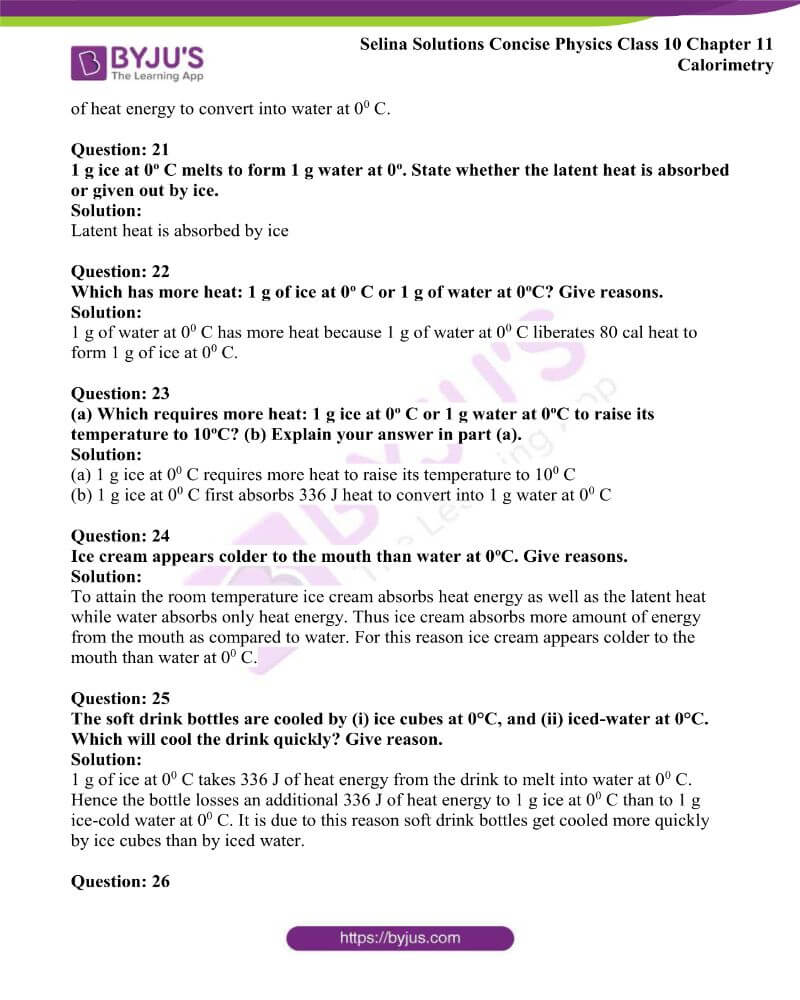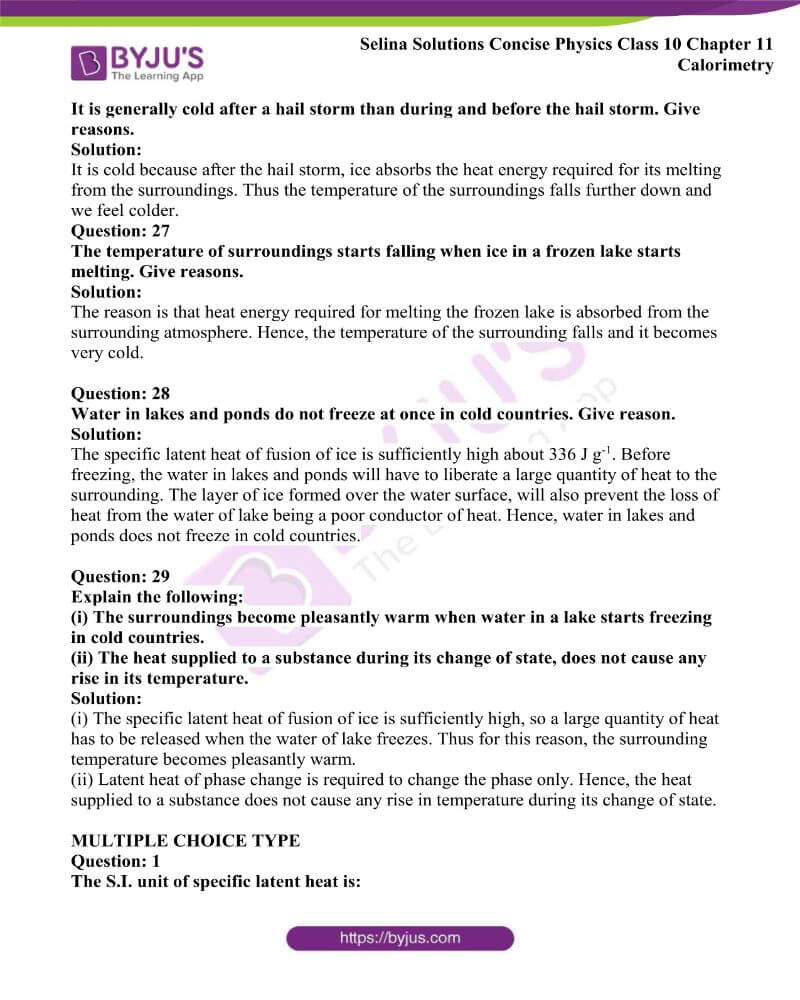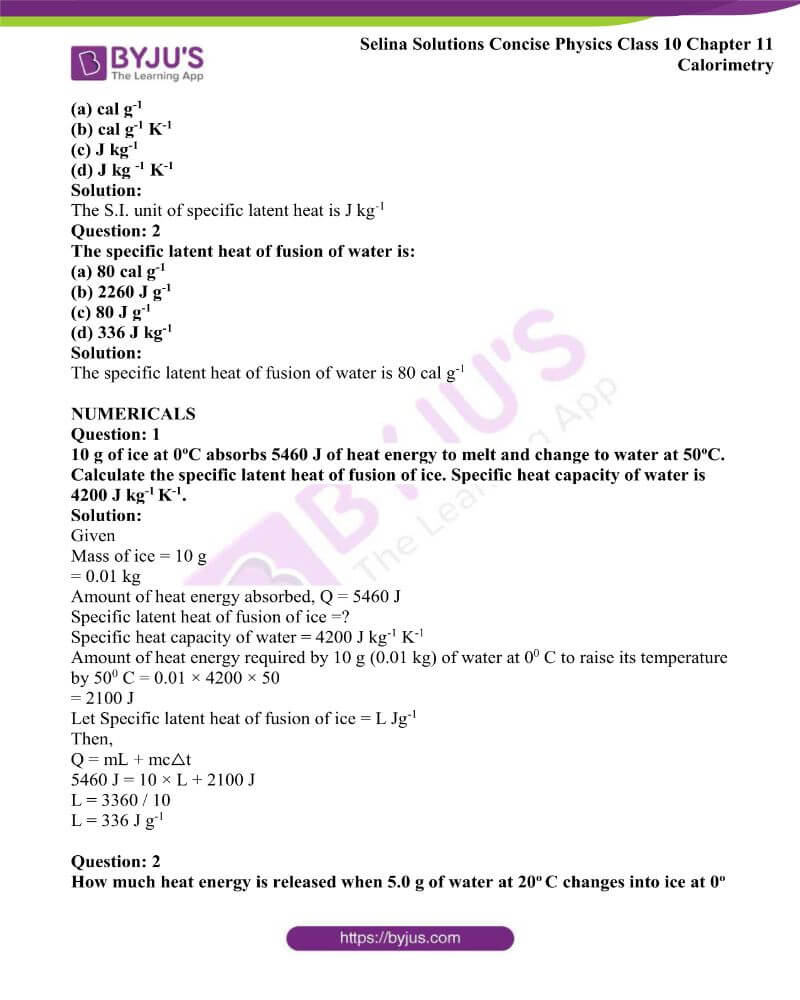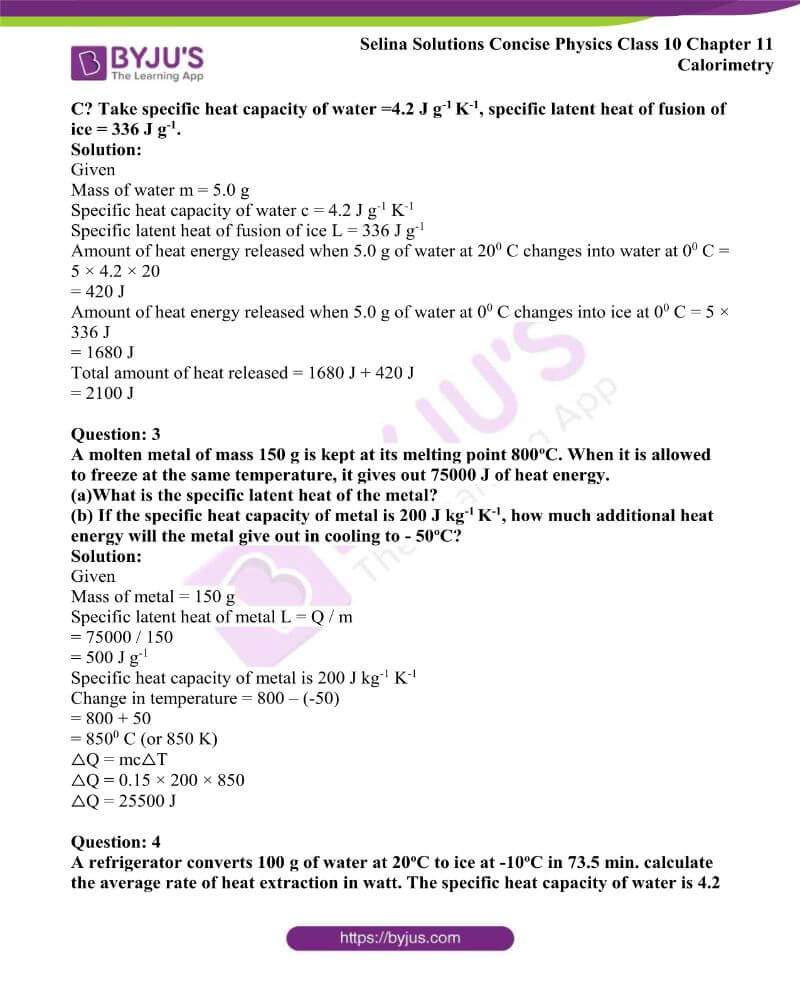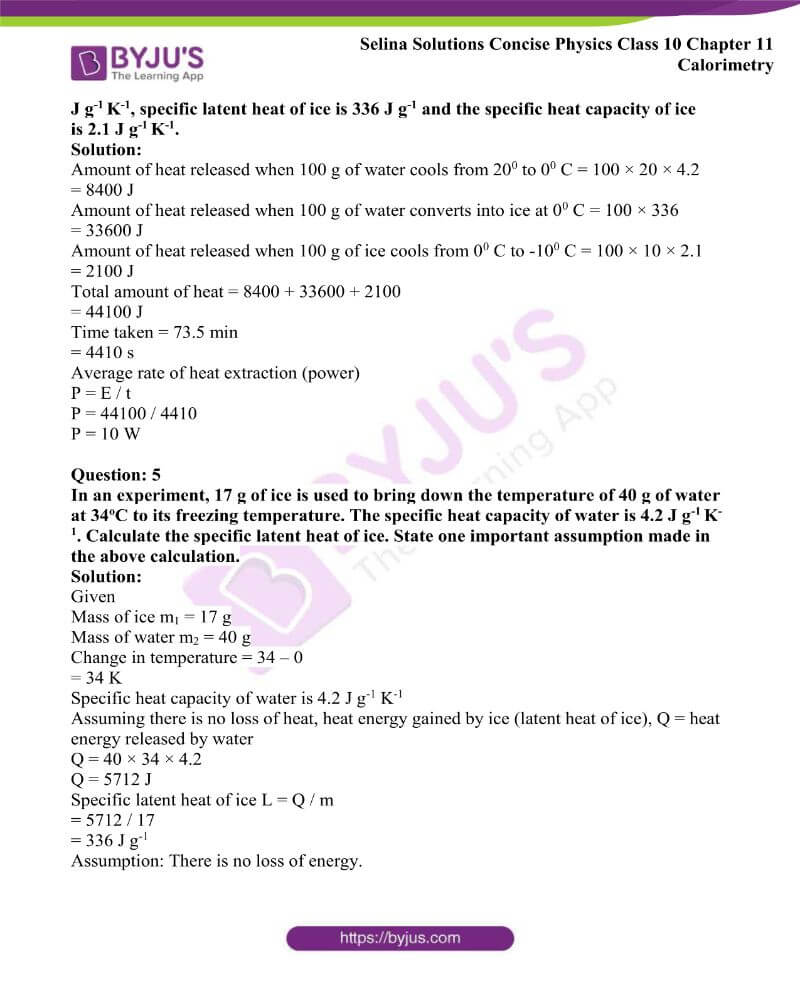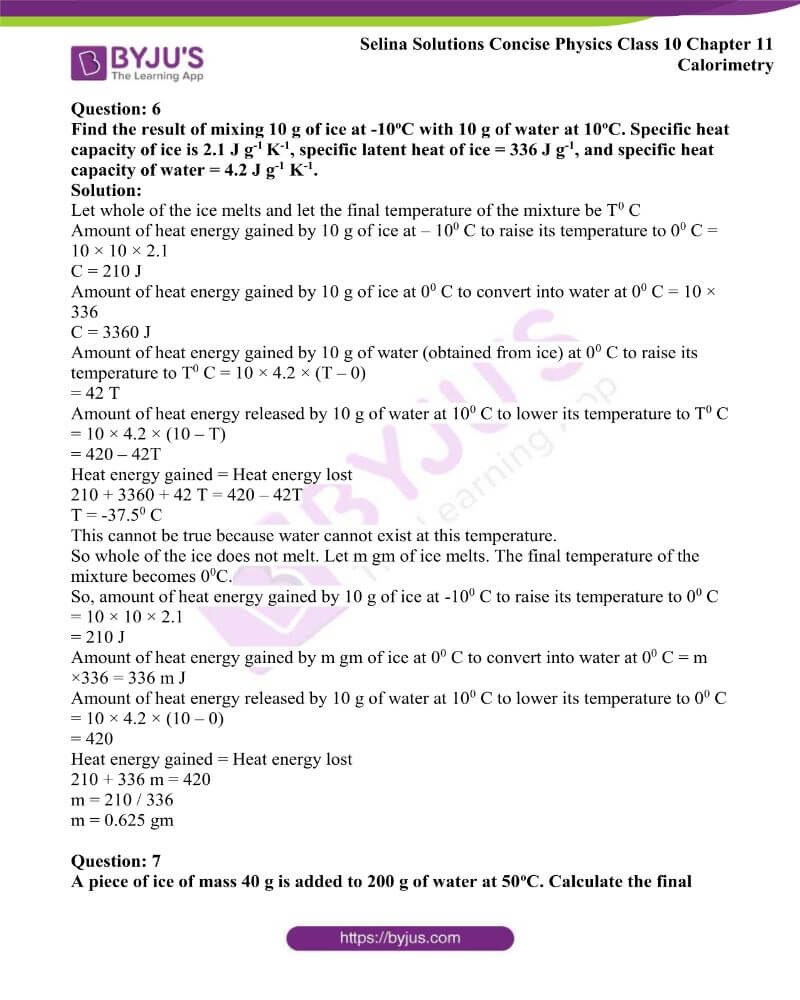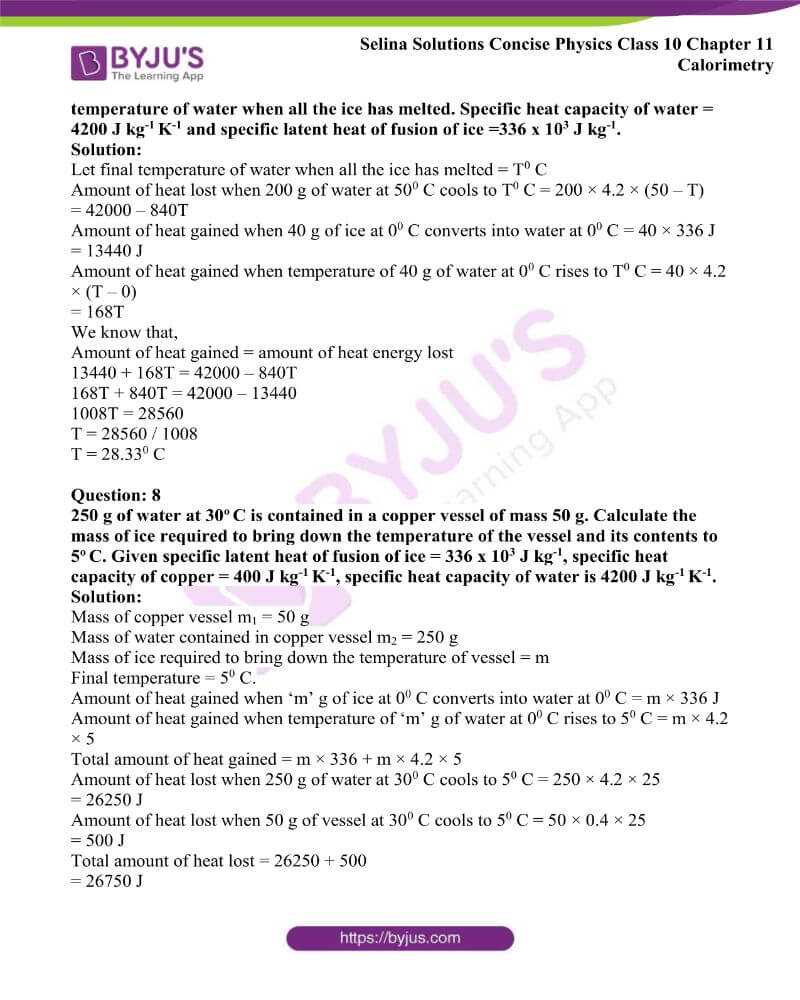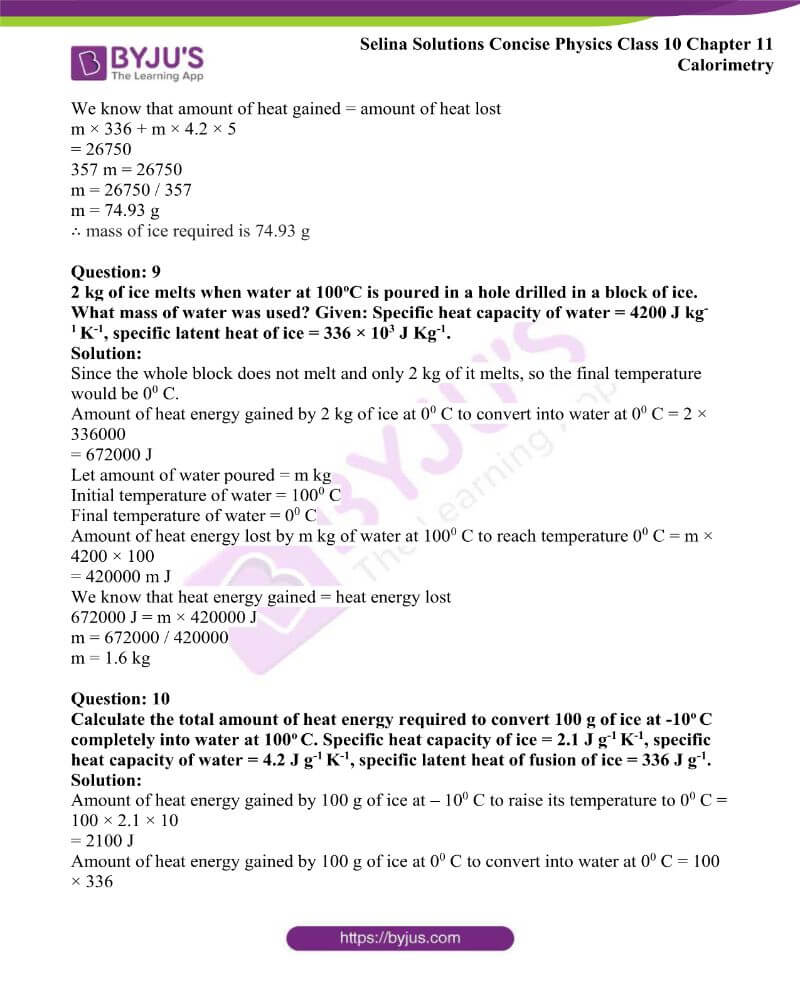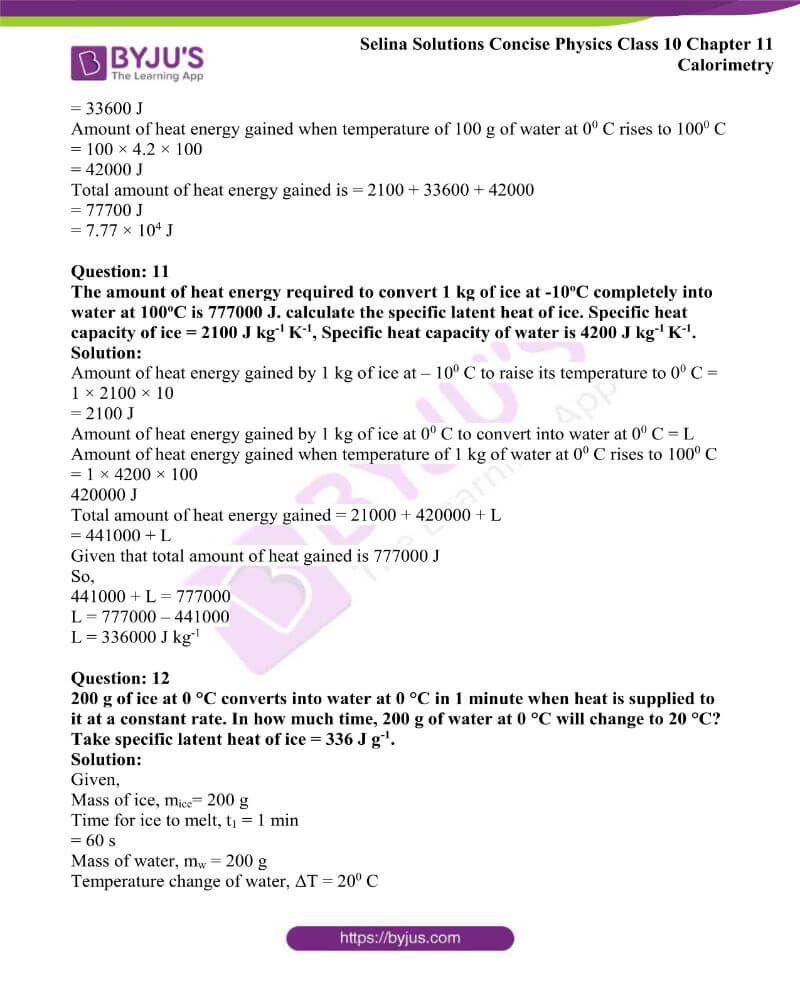### Access Answers to Physics Selina Solutions Concise Physics Class 10 Chapter 11 Calorimetry

Exercise 11(A) page no: 269

Question: 1

Define the term heat.

Solutions:

The term heat is defined as the internal energy of molecules constituting the body. It flows from a hot body to a cold body when they are kept in contact.

Question: 2

Name the S.I. unit of heat.

Solution:

Joule is the S.I. unit of heat.

Question: 3

Define the term calorie. How is it related to joule?

Solution:

One calorie is the heat energy required to raise the temperature of 1 g of water from 14.50 C to 15.50 C. The unit calorie is related to the S.I. unit joule as follows:

1 calorie = 4.186 J.

Question: 4

Define one kilo-calorie of heat.

Solution:

One kilo-calorie is the heat energy required to raise the temperature of 1 kg of water from 14.50 C to 15.50 C

Question: 5

Define temperature and name its S.I. unit.

Solution:

Temperature is defined as the quantity which determines the direction of flow of heat when two bodies at different temperatures are placed in contact. The S.I. unit of temperature is Kelvin (K).

Question: 6

State three differences between heat and temperature.

 Heat Temperature Heat is a form of internal energy obtained due to random motion and attractive force of molecules in a substance. Temperature is a quantity which determines the direction of flow of heat on keeping the two bodies at different temperatures in contact. The S.I. unit of heat is joule (J) The S.I. unit of temperature is Kelvin (K) Heat is measured by the principle of calorimetry Temperature is measured by a thermometer.

Question: 7

Define calorimetry.

Solution:

The measurement of the quantity of heat is known as calorimetry.

Question: 8

Define the term heat capacity and state its S.I. unit.

Solution:

The term heat capacity of a body is the amount of heat energy required to raise its temperature by 10C or 1 K. The S.I. unit of heat capacity is joule per kelvin (JK-1)

Question: 9

Define the term specific heat capacity and state its S.I. unit.

Solution:

The term specific heat capacity of a substance is the amount of heat energy required to raise the temperature of a unit mass of that substance through 10C (or 1 K). The S.I. unit of specific heat capacity is joule per kilogram per kelvin (J kg-1 K-1).

Question: 10

How is the heat capacity of a body related to the specific heat capacity of its substance?

Solution:

The equation which relates the heat capacity of a body to the specific heat capacity of its substance is

Heat capacity = Mass × Specific heat capacity

Question: 11

State three differences between the heat capacity and specific heat capacity.

Solution:

 Heat capacity Specific heat capacity Heat capacity is the amount of heat energy required to raise the temperature of the entire body by 10 C Specific heat capacity is the amount of heat energy required to raise the temperature of a unit mass of the body by 10C It depends both on the substance and mass of the body. More the mass of the body, more is its heat capacity It does not depend on the mass of the body S.I. unit is J K-1 S.I. unit is J kg-1 K-1

Question: 12

Name a liquid which has the highest specific heat capacity.

Solution:

A liquid which has the highest specific heat capacity is water.

Question: 13

Write the approximate value of specific heat capacity of water in S.I. unit.

Solution:

The specific heat capacity of water is 4200 J kg-1 K-1.

Question: 14

What do you mean by the following statements:

(i) The heat capacity of a body is 50 JK-1?

(ii) The specific heat capacity of copper is 0.4 J g-1 K-1?

Solution:

(i) The statement heat capacity of a body is 50 JK-1 means we have to supply 50 joules of energy to increase the temperature of this body by 1 K.

(ii) The statement specific heat capacity of copper is 0.4 J g-1 K-1 means we have to supply 0.4 joules of energy to increase the temperature of one gram of copper by 1 K.

Question: 15

Specific heat capacity of a substance A is 3.8 J g-1 K-1 and of substance B is 0.4 J g-1 k-1. Which substance is a good conductor of heat? How did you arrive at your conclusion?

Solution:

The specific heat capacity of a substance B is lower than that of A. So, the rise in temperature for B will be more than that of A for same mass and same heat energy. Less is the specific heat capacity of a substance, the better it acts as a good conductor. Thus substance B is a good conductor of heat than the substance A.

Question: 16

Name two factors on which the heat energy liberated by a body on cooling depends.

Solution:

The factors on which the heat energy liberated by a body on cooling depends are as follows:

(i) Mass

(ii) Specific heat capacity

(iii) Temperature of the body

Question: 17

Name three factors on which the heat energy absorbed by a body depends and state how does it depend on them.

Solution:

The three factors on which the heat energy absorbed by a body depends are

(i) The mass of the body: The amount of heat energy required is directly proportional to the mass of the object.

(ii) The increase in temperature of the body: The amount of heat energy required is directly proportional to the rise in temperature.

(iii) The material of the body: The amount of heat energy required depends on the substance of the object which is expressed in terms of its specific heat capacity c.

Question: 18

Write the expression for the heat energy Q received by m kg of a substance of specific heat capacity c J kg-1 K-1 when it is heated through △ to C.

Solution:

The expression for the heat energy Q is given by

Q = c m △t (in joule)

Question: 19

Same amount of heat is supplied to two liquids A and B. The liquid A shows a greater rise in temperature. What can you say about the heat capacity of A as compared to that of B?

Solution:

The substance with low specific heat capacity shows a rapid and high rise in temperature. Hence, the heat capacity of liquid A is less than that of B.

Question: 20

Two blocks P and Q of different metals having their mass in the ratio 2:1 are given the same amount of heat. Their temperatures rise by the same amount, compare their specific heat capacities.

Solution:

Let Cp and Cq be the specific heat capacities of blocks P and Q respectively

We know that,

C = Q / m × △t

Now,

Cp / Cq = (Q / 2m × △t) / (Q / m × △t)

Cp / Cq = (Q / 2m × △t) × (m × △t / Q)

Cp / Cq = 1 / 2

Therefore the required ratio is 1: 2

Question: 21

What is the principle of the method of mixture? What other name is given to it? Name the law on which this principle is based.

Solution:

Heat energy lost by the hot body = Heat energy gained by the cold body. This is called the principle of the method of mixture. The other name to it is the principle of calorimetry. This principle is based on the law of conservation of energy.

Question: 22

A mass m1 of a substance of specific heat capacity c1 at temperature T1 is mixed with a mass m2 of other substance of specific heat capacity c2 at a lower temperature T2. Deduce the expression for the temperature t of the mixture. State the assumption made, if any.

Solution:

A mass m1 of a substance A of specific heat capacity c1 at temperature T1 is mixed with a mass m2 of other substance B of specific heat capacity c2 at a lower temperature T2 and final temperature of the mixture becomes T.

Fall in temperature of substance A = T1 – T

Rise in temperature of substance B = T – T2

Heat energy lost by A = m1 × c1 × fall in temperature = m1c1 (T1 – T)

Heat energy gained by B = m2 × c2 × rise in temperature = m2c2 (T – T2)

If no energy lost in the surrounding, then by the principle of mixtures,

Heat energy lost by A = Heat energy gained by B

m1c1 (T1 – T) = m2c2 (T – T2)

We get after rearranging this equation

T = (m1c1 T1 + m2c2 T2) / m1c1 + m2c2

We have assumed here that there is no loss of heat energy.

Question: 23

Why do the farmers fill their fields with water on a cold winter night?

Solution:

On a cold winter night, if the atmospheric temperature falls below 00 C, water in the fine capillaries of plants will freeze, so the veins will burst due to the increase in volume of water on freezing. As a result, plants will die and the crop will get destroyed. In order to save crop on such cold nights, farmers fill their fields with water because water has a high specific heat capacity, so it does not allow the temperature in the surrounding area of plants to fall up to 00 C.

Question: 24

Discuss the role of high specific heat capacity of water with reference to climate in coastal areas.

Solution:

The specific heat capacity of water is very high about five times as high as that of sand. Hence the heat energy required for the same rise in temperature by a certain mass of water will be nearly five times than that required by the same mass of sand. Similarly, a certain mass of water will impart nearly five times more heat energy than that given by the same mass of sand for the same fall in temperature. As such sand gets heated or cooled more rapidly as compared to water under the similar conditions. Thus a large difference in temperature is developed between the land and the sea due to which land and sea breezes are formed. The climate near the seashore becomes moderate by these breezes.

Question: 25

Water is used in hot water bottles for fomentation. Give reason.

Solution:

Water does not cool quickly due to its high specific heat capacity, so a hot water bottle provides more heat energy for fomentation over a longer period. Hence water is used in hot water bottles for fomentation.

Question: 26

What property of water makes it an effective coolant?

Solution:

By allowing water to flow in pipes around the heated parts of a machine, heat energy from such parts is removed. Water in pipes can extract more heat from the surroundings without much rise in its temperature because of its high specific heat capacity. For this reason, water is used as an effective coolant.

Question: 27

Give one example each where high specific heat capacity of water is used (i) as coolant, (ii) as heat reservoir.

Solution:

(i) Radiators in car and generator use water as coolant

(ii) Water is used as a heat reservoir for wine and juice bottles to avoid their freezing.

Question: 28

A liquid X has specific heat capacity higher than the liquid Y. Which liquid is useful as (i) coolant in car radiators and, (ii) heat reservoir to keep juice bottles without freezing?

Solution:

As the specific heat capacity of liquid X is higher than the liquid Y, so for same mass and same heat energy, the rise in temperature for X will be less than that of Y.

(i) The liquid needs to absorb more energy without much change in temperature as a coolant in car radiators. Hence liquid X is useful for this function.

(ii) The liquid needs to give out a large amount of heat before reaching freezing temperatures as a heat reservoir to keep juice bottles without freezing. Hence, liquid X is useful for this function.

Question: 29

(a) What is calorimeter?

(b)Name the material of which it is made of. Give two reasons for using the material stated by you.

(c) Out of the three metals A, B and C of specific heat 900 J kg-1 °C-1, 380 J kg-1 °C-1 and 460 J kg-1 °C-1 respectively, which will you prefer for calorimeter? Given reason.

(d) How is the loss of heat due to radiation minimised in a calorimeter?

Solution:

(a) A cylindrical vessel which is used to measure the amount of heat gained or lost by a body when it is mixed with the other body is called a calorimeter.

(b) It is made up of a thin sheet of copper because

(i) Copper is a good conductor of heat, so the vessel soon acquires the temperature of its contents.

(ii) Copper has the low specific heat capacity so the heat capacity of the calorimeter is low and the amount of heat energy taken by the calorimeter from the contents to acquire its temperature, is very small.

(c) Heat capacity of the calorimeter should be less. Thus out of three metals the one which has the lowest specific heat capacity should be preferred.

(d) The loss of heat due to radiation can be minimised in a calorimeter by polishing the outer and inner surface of the vessel.

Question: 30

Why the base of a cooking pan made thick and heavy?

Solution:

The base of a cooking pan is made thick and heavy because its heat capacity becomes large due to which it gets heated slowly and it imparts sufficient heat energy at a slow rate to the food for its proper cooking. Further after cooking it keeps the food warm for a long time.

MULTIPLE CHOICE TYPE

Question: 1

The S.I. unit of heat capacity is:

(a) J kg-1

(b) J K-1

(c) J kg-1 K-1

(d) cal 0C-1

Solution:

The S.I. unit of heat capacity is (b) J K-1

Question: 2

The S.I. unit of specific heat capacity is:

(a) J kg-1

(b) J K-1

(c) J kg-1 K-1

(d) kcal kg-10C-1

Solution:

The S.I. unit of specific heat capacity is (c) J kg-1 K-1

Question: 3

The specific heat capacity of water is:

(a) 4200 J kg-1 K-1

(b) 420 J g-1 K-1

(c) 0.42 J g-1 K-1

(d) 4.2 J kg-1 K-1

Solution:

The specific heat capacity of water is (a) 4200 J kg-1 K-1

NUMERICAL

Question: 1

By imparting heat to a body, its temperature rises by 150C. What is the corresponding rise in temperature on the Kelvin scale?

Solution:

The size of 1 degree on the Kelvin scale and the size of 1 degree on the Celsius scale are equal. Hence, on both the Kelvin and Celsius scales the difference or change in temperature is the same. Therefore the corresponding rise in temperature on the Kelvin scale will be 15 K

Question: 2

(a)Calculate the heat capacity of a copper vessel of mass 150 g if the specific heat capacity of copper is 410 J kg-1 K-1.

(b)How much heat energy will be required to increase the temperature of the vessel in part (a) from 25oC to 35oC?

Solution:

(a) Given

Mass of copper vessel = 150 g

= 0.15 kg

The specific heat capacity of copper = 410 J kg-1 K-1

We know that,

Heat capacity = Mass × specific heat capacity

= 0.15 kg × 410 J kg-1 K-1

= 61.5 JK-1

Change in temperature = (35 – 25)0 C

= 100 C

= 10 K

(b) The energy required to increase the temperature of vessel

△Q = mc△T

△Q = 0.15 × 410 × 10

△Q = 615 J

Question: 3

A piece of iron of mass 2.0 kg has a heat capacity of 966 J K-1. Find

(i) Heat energy needed to warm it by 15oC, and

(ii) Its specific heat capacity in S.I unit.

Solution:

(i) We know that,

Heat energy needed to raise the temperature by 150 C = heat capacity × change in temperature

Heat energy required = 966 J K-1 × 15 K

Heat energy required = 14490 J

(ii) We know that,

Specific heat capacity = heat capacity / mass of substance

Specific heat capacity = 966 / 2

Specific heat capacity = 483 J kg-1 K-1

Question: 4

Calculate the amount of heat energy required to raise the temperature of 100 g of copper from 20oC to 70oC. Specific heat of capacity of copper =390 J kg-1 K-1.

Solution:

Given

Mass of copper m = 100 g

= 0.1 kg

Change of temperature △t = (70 – 20)0 C

= 500 C

Specific heat capacity of copper = 390 J kg-1 K-1

Amount of heat required to raise the temperature of 0.1 kg of copper is

Q = m × △t × c

Q = 0.1 × 50 × 390

Q = 1950 J

Question: 5

1300 J of heat energy is supplied to raise the temperature of 0.5 kg of lead from 20oC to 40oC. Calculate the specific heat capacity of lead.

Solution:

Given

Heat energy supplied = 1300 J

Mass of lead = 0.5 kg

Change in temperature = (40 – 20)0 C

= 200 C

C = △Q / m△T

C = 1300 / 0.5 × 20

C = 130 J kg-1 K-1

Question: 6

Find the time taken by a 500 W  heater to raise the temperature of 50 kg of material of specific heat capacity 960 J kg-1 K-1, from 18oC to 38oC. Assume that all the heat energy supplied by heater is given to the material.

Solution:

Specific heat capacity of material c = 960 J kg-1 K-1

Change in temperature △T = (38 – 18)0 C

= 200 C or 20 K

Power of heater P = 500 W

△Q = mc△T

△Q = 50 × 960 × 20

Time taken by a heater to raise the temperature of material

t = △Q / P

t = (50 × 960 × 20) / 500

t = 1920 seconds

t = 32 minutes

Question: 7

An electric heater of power 600 W raises the temperature of 4.0 kg of a liquid from 10.0 0C to 15.0 0C in 100 s. Calculate:

(i) the heat capacity of 4.0 kg of liquid, and

(ii) the specific heat capacity of liquid

Solution:

Power of heater P = 600 W

Mass of liquid m = 4.0 kg

Change in temperature of liquid = (15 – 10)0C

= 50 C (or 5 K)

Time taken to raise its temperature = 100 s

Heat energy required to heat the liquid

△Q = mc△T and

△Q = P × t

△Q = 600 × 100

△Q = 60000 J

c = △Q / m△T

c = 60000 / (4 × 5)

c = 3000 J kg-1 K-1

= 3 × 103 J kg-1 K-1

Heat capacity = c × m

Heat capacity = 4 × 3000 J kg-1 K-1

Heat capacity = 1.2 × 104 J / K

Question: 8

0.5 kg of lemon squash at 30o C is placed in a refrigerator which can remove heat at an average rate of 30 J s-1. How long will it take to cool the lemon squash to 5o C? Specific heat capacity of squash = 4200 J kg-1 K-1.

Solution:

Change in temperature = 30 – 5

= 25 K

△Q = mc△T

△Q = 0.5 × 4200 × 25

△Q = 52500 J

t = △Q / P

t = 52500 / 30

t = 1750 s

t = 29 min 10 sec

Question: 9

A mass of 50 g of a certain metal at 150° C is immersed in 100 g of water at 11° C. The final temperature is 20° C. Calculate the specific heat capacity of the metal. Assume that the specific heat capacity of water is 4.2 J g-1 K-1.

Solution:

Heat liberated by metal = m × s × △t

= 50 × s × (150 – 20)

Heat absorbed by water = mw × sw × △t

= 100 × 4.2 × (20 – 11)

Heat energy lost = heat energy gained

50 × s × (150 – 20) = 100 × 4.2 × (20 – 11)

s = 0.582 J g-1 K-1

Question: 10

45 g of water at 50o C in a beaker is cooled when 50 g of copper at 18oC is added to it. The contents are stirred till a final constant temperature is reached. Calculate the final temperature. The specific heat capacity of copper is 0.39 J g-1 K-1 and that of water is 4.2 J g-1 K-1. State the assumptions used.

Solution:

Mass of water (m1) = 45 g

Temperature of water (T1) = 500 C

Mass of copper (m2) = 50 g

Temperature of copper (T2) = 180 C

Final temperature (T) =?

The specific heat capacity of the copper c2 = 0.39 J / g / K

The specific heat capacity of water c1 = 4.2 J / g / K

m1c1 (T1 – T) = m2c2 (T – T2)

T = (m1c1T1 + m2c2T2) / (m2c2 + m1c1)

T = (45 × 4.2 × 50) + (50 × 0.39 × 18) / (45 × 4.2) + (50 × 0.39)

T = (9450 + 351) / (189 + 19.5)

T = 9801 / 208.5

T = 470 C

Question: 11

200 g of hot water at 80o C is added to 300 g of cold water at 10oC. Neglecting the heat taken by the container, calculate the final temperature of the mixture of water. Specific heat capacity of water = 4200 J kg-1 K-1.

Solution:

Mass of hot water (m1) = 200 g

Temperature of hot water (T1) = 800 C

Mass of cold water (m2) = 300 g

Temperature of cold water (T2) = 100 C

Final temperature (T) =?

m1c1 (T1 – T) = m2c2 (T – T2)

c1 = c2

T = m1T1 + m2T2 / m2 + m1

T = [(200 × 80) + (300 × 10)] / 500

T = (16000 + 3000) / 500

T = 19000 / 500

T = 380 C

Question: 12

The temperature of 600 g of cold water rises by 15o C when 300 g of hot water at 50o C is added to it. What was the initial temperature of the cold water?

Solution:

Mass of hot water (m1) = 300 g

Temperature (T1) = 500 C

Mass of cold water (m2) = 600 g

Change in temperature of cold water (T – T2) = 150 C

Final temperature = T0 C

The specific heat capacity of water is c

m1c (T1 – T) = m2c (T – T2)

300 (50 – T) = 600 (15)

T = 6000 / 300

T = 200 C

Final temperature = 200 C

Change in temperature = 150 C

Initial temperature of cold water = 200 C – 150 C

= 50 C

Question: 13

1.0 kg of water is contained in a 1.25 kW kettle. Calculate the time taken for the temperature of water to rise from 25° C to its boiling point 100° C. Specific heat capacity of water = 4.2 J g-1 K-1.

Solution:

Heat energy = Power × time

Heat energy contained by water = 1000 × 4.2 × 75

1000 × 4.2 × 75 = 1250 × time

time = (1000 × 4.2 × 75) / 1250

time = 315000 / 1250

time = 252 sec

time = 4 min 12 sec

Exercise 11(B) page no: 280

Question: 1

(a)What do you understand by the change of phase of substance?

(b)Is there any change in temperature during the change of phase?

(c)Does the substance absorb or liberate any heat during the change of phase?

(d) What is the name given to the energy absorbed during a phase change?

Solution:

(a) The change of phase is a process of change from one state to another at a constant temperature.

(b) No, there is no change in temperature during the change of phase.

(c) Yes, the substance absorb or liberates heat during the change of phase.

(d) The name given to the energy absorbed during a phase change is latent heat.

Question: 2

A substance changes from its solid state to the liquid state when heat is supplied to it

a. Name the process.

b. What name is given to heat observed by the substance.

c. How does the average kinetic energy of the molecules of the substance change.

Solution:

(a) This process is known as melting.

(b) The name given to heat observed by the substance is the latent heat of melting.

(c) The average kinetic energy of the molecules does not change as there is no change in temperature.

Question: 3

A substance on heating undergoes (i) a rise in its temperature, (ii) A change in its phase without change in its temperature. In each case, state the change in energy of molecules of the substance.

Solution:

(i) The average kinetic energy of molecules increases.

(ii) Average potential energy of molecules increases.

Question: 4

How does the (a) average kinetic energy (b) average potential energy of molecules of a substance change during its change in phase at a constant temperature, on heating?

Solution:

(a) The average kinetic energy of molecules does not change.

(b) Average potential energy of molecules increases.

Explanation: The heat supplied when a substance is heated at constant temperature i.e., during its phase change state makes the vibrating molecules gain potential energy to overcome the intermolecular force of attraction and move about freely. This means that the substance changes its form.

However, this heat does not increase the kinetic energy of the molecules. So, there is no rise in temperature during the change in phase of a substance.

This heat supplied to the substance is called as latent heat and is utilized in changing the state of matter without increase in temperature.

Question: 5

State the effect of the presence of impurity on the melting point of ice. Give one use of it.

Solution:

The melting point of a substance decreases by the presence of impurities in it.

Use: This is used in making the freezing mixture by adding salt to ice. The freezing mixture is used in preparing kulfis.

Question: 6

State the effect of the increase of pressure on the melting point of ice.

Solution:

By the increase in pressure, the melting point of ice decreases. The melting point of ice decreases by 0.00720 C for every one-atmosphere rise in pressure.

Question: 7

The diagram shows the change of phases of a substance on a temperature-time graph on heating the substance at a constant rate.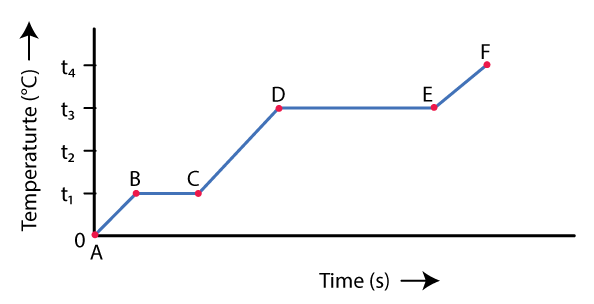(a)What do parts AB, BC, CD and DE represent?

(b) What is the melting point of the substance?

(c) What is the boiling point of the substance?

Solution:

(a) AB part shows the rise in temperature of solid from 00 C to t10 C

BC part shows melting at temperature t10 C

CD part shows a rise in temperature of liquid from t10 C to t30 C

DE part shows the boiling at temperature t30 C

(b) The melting point of the substance is t10 C

(c) The boiling point of the substance is t30 C

Question: 8

1 kg of ice at 0o is heated at a constant rate and its temperature is recorded after every 30 seconds till steam is formed at 100o C. Draw a temperature-time graph to represent the change of phases.

Solution: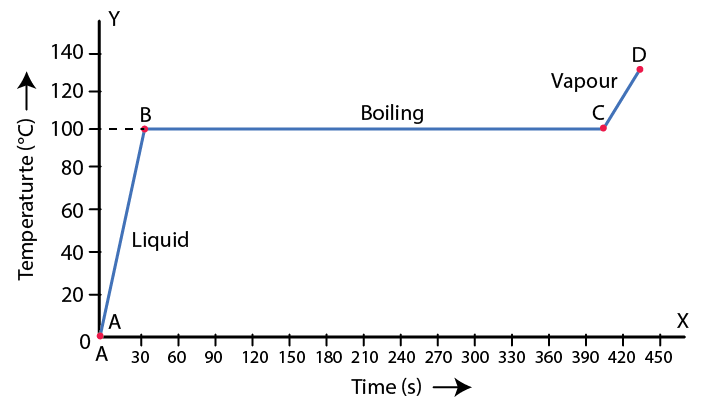Question: 9

Explain the terms boiling and boiling point.

Solution:

The change from liquid to gas phase on absorption of heat at a constant temperature is called boiling.

The particular temperature at which vaporization occurs is known as the boiling point of liquid.

Question: 10

How is the volume of water affected when it boils at 100oC?

Solution:

Volume of water increases when it boils at 1000 C

Question: 11

How is the boiling point of water affected when some salt is added to it?

Solution:

The boiling point of water increases by addition of salt to it. When common salt is added to water, it boils at a temperature higher than 1000 C. Cooking becomes easier and faster as the salt in water provides sufficient heat energy to its contents before boiling.

Question: 12

What is the effect of increase in pressure on the boiling point of a liquid?

Solution:

The boiling point of a liquid increases with the increase in pressure.

Question: 13

Water boils at 120 °C in a pressure cooker. Explain the reason

Solution:

The boiling point of liquid increases with the increase in pressure and decreases with the decrease in pressure. At one atmospheric pressure, the boiling point of pure water is 1000 C. In a pressure cooker, steam is not allowed to escape out. The vapour pressure on water inside the cooker becomes nearly 1.75 times the atmospheric pressure. Thus water boils at about 1200 C to 1250 C due to increase in pressure.

Question: 14

Write down the approximate range of temperature at which water boils in a pressure cooker.

Solution:

The water boils at about 1200 C to 1250 C in a pressure cooker.

Question: 15

It is difficult to cook vegetables on hills and mountains. Explains the reason.

Solution:

Atmospheric pressure is low at high altitudes. Hence boiling point of water decreases and so it does not provide the required heat energy to its contents for cooking. Due to this reason it becomes difficult to cook vegetables on hills and mountains.

Question: 16

Complete the following sentences:

(a)When ice melts, its volume………….

(b)Decrease in pressure over ice ………….. its melting point.

(c)Increase in pressure ………..the boiling point of water.

(d)A pressure cooker is based on the principle that boiling point of water increases with the …………..

(e)The boiling point of water is defined as ………………………..

(f) Water can be made to boil at 115°C by…………….. pressure over its surface.

Solution:

(a) When ice melts, its volume decreases.

(b) Decrease in pressure over ice increases its melting point.

(c) Increase in pressure increases the boiling point of water.

(d) A pressure cooker is based on the principle that the boiling point of water increases with the increase in pressure.

(e) The boiling point of water is defined as the constant temperature at which water changes to steam.

(f) Water can be made to boil at 1150 C by increasing pressure over its surface.

Question: 17

What do you understand by the term latent heat?

Solution:

Heat energy absorbed or liberated in a change of phase is not externally manifested by any rise or fall in temperature is called the latent heat.

Question: 18

Define the term specific latent heat of fusion of ice. State its S.I. unit.

Solution:

The specific latent heat of fusion of ice is the heat energy required to melt a unit mass of ice at 00 C to water at 00 C without any change in temperature.

Question: 19

Write the approximate value of specific latent heat of ice.

Solution:

The approximate value of specific latent heat of ice is 336000 J kg-1

Question: 20

‘The specific latent heat of fusion of ice is 336 J g-1 ‘. Explain the meaning of this statement.

Solution:

The specific latent heat of fusion of ice is 336 J g-1 means 1 g of ice at 00 C absorbs 336 J of heat energy to convert into water at 00 C.

Question: 21

1 g ice at 0o C melts to form 1 g water at 0o. State whether the latent heat is absorbed or given out by ice.

Solution:

1 g ice at 0o C melts to form 1 g water at 0o. In this situation, the latent heat is absorbed by the ice.

Question: 22

Which has more heat: 1 g of ice at 0o C or 1 g of water at 0oC? Give reasons.

Solution:

1 g of water at 00 C has more heat because 1 g of water at 00 C liberates 80 cal heat to form 1 g of ice at 00 C.

Question: 23

(a) Which requires more heat: 1 g ice at 0o C or 1 g water at 0oC to raise its temperature to 10oC? (b) Explain your answer in part (a).

Solution:

(a) 1 g ice at 00 C requires more heat to raise its temperature to 100 C

(b) 1 g ice at 00 C first absorbs 336 J heat to convert into 1 g water at 00 C

Question: 24

Ice cream appears colder to the mouth than water at 0oC. Give reasons.

Solution:

To attain the room temperature ice cream absorbs heat energy as well as the latent heat while water absorbs only heat energy. Thus ice cream absorbs more amount of energy from the mouth as compared to water. For this reason ice cream appears colder to the mouth than water at 00 C.

Question: 25

The soft drink bottles are cooled by (i) ice cubes at 0°C, and (ii) iced-water at 0°C. Which will cool the drink quickly? Give reason.

Solution:

1 g of ice at 00 C takes 336 J of heat energy from the drink to melt into water at 00 C. Hence the bottle losses an additional 336 J of heat energy to 1 g ice at 00 C than to 1 g ice-cold water at 00 C. It is due to this reason soft drink bottles get cooled more quickly by ice cubes than by iced water.

Question: 26

It is generally cold after a hail storm than during and before the hail storm. Give reasons.

Solution:

It is cold because after the hail storm, ice absorbs the heat energy required for its melting from the surroundings. Thus the temperature of the surroundings falls further down and we feel colder.

Question: 27

The temperature of surroundings starts falling when ice in a frozen lake starts melting. Give reasons.

Solution:

The reason is that heat energy required for melting the frozen lake is absorbed from the surrounding atmosphere. Hence, the temperature of the surrounding falls and it becomes very cold.

Question: 28

Water in lakes and ponds do not freeze at once in cold countries. Give reason.

Solution:

The specific latent heat of fusion of ice is sufficiently high about 336 J g-1. Before freezing, the water in lakes and ponds will have to liberate a large quantity of heat to the surrounding. The layer of ice formed over the water surface, will also prevent the loss of heat from the water of lake being a poor conductor of heat. Hence, water in lakes and ponds does not freeze in cold countries.

Question: 29

Explain the following:

(i) The surroundings become pleasantly warm when water in a lake starts freezing in cold countries.

(ii) The heat supplied to a substance during its change of state, does not cause any rise in its temperature.

Solution:

(i) The specific latent heat of fusion of ice is sufficiently high, so a large quantity of heat has to be released when the water of lake freezes. Thus, for this reason, the surrounding temperature becomes pleasantly warm.

(ii) Latent heat of phase change is required to change the phase only. Hence, the heat supplied to a substance does not cause any rise in temperature during its change of state.

MULTIPLE CHOICE TYPE

Question: 1

The S.I. unit of specific latent heat is:

(a) cal g-1

(b) cal g-1 K-1

(c) J kg-1

(d) J kg -1 K-1

Solution:

The S.I. unit of specific latent heat is (c) J kg-1

Question: 2

The specific latent heat of fusion of water is:

(a) 80 cal g-1

(b) 2260 J g-1

(c) 80 J g-1

(d) 336 J kg-1

Solution:

The specific latent heat of fusion of water is (a) 80 cal g-1

NUMERICAL

Question: 1

10 g of ice at 0oC absorbs 5460 J of heat energy to melt and change to water at 50oC. Calculate the specific latent heat of fusion of ice. Specific heat capacity of water is 4200 J kg-1 K-1.

Solution:

Given

Mass of ice = 10 g

= 0.01 kg

Amount of heat energy absorbed, Q = 5460 J

Specific latent heat of fusion of ice =?

Specific heat capacity of water = 4200 J kg-1 K-1

Amount of heat energy required by 10 g (0.01 kg) of water at 00 C to raise its temperature by 500 C = 0.01 × 4200 × 50

= 2100 J

Let Specific latent heat of fusion of ice = L Jg-1

Then,

Q = mL + mc△t

5460 J = 10 × L + 2100 J

L = 3360 / 10

L = 336 J g-1

Question: 2

How much heat energy is released when 5.0 g of water at 20o C changes into ice at 0o C? Take specific heat capacity of water =4.2 J g-1 K-1, specific latent heat of fusion of ice = 336 J g-1.

Solution:

Given

Mass of water m = 5.0 g

Specific heat capacity of water c = 4.2 J g-1 K-1

Specific latent heat of fusion of ice L = 336 J g-1

Amount of heat energy released when 5.0 g of water at 200 C changes into water at 00 C = 5 × 4.2 × 20

= 420 J

Amount of heat energy released when 5.0 g of water at 00 C changes into ice at 00 C = 5 × 336 J

= 1680 J

Total amount of heat released = 1680 J + 420 J

= 2100 J

Question: 3

A molten metal of mass 150 g is kept at its melting point 800oC. When it is allowed to freeze at the same temperature, it gives out 75000 J of heat energy.

(a)What is the specific latent heat of the metal?

(b) If the specific heat capacity of metal is 200 J kg-1 K-1, how much additional heat energy will the metal give out in cooling to – 50oC?

Solution:

Given

Mass of metal = 150 g

Specific latent heat of metal L = Q / m

= 75000 / 150

= 500 J g-1

Specific heat capacity of metal is 200 J kg-1 K-1

Change in temperature = 800 – (-50)

= 800 + 50

= 8500 C (or 850 K)

△Q = mc△T

△Q = 0.15 × 200 × 850

△Q = 25500 J

Question: 4

A refrigerator converts 100 g of water at 20oC to ice at -10oC in 73.5 min. calculate the average rate of heat extraction in watt. The specific heat capacity of water is 4.2 J g-1 K-1, specific latent heat of ice is 336 J g-1 and the specific heat capacity of ice is 2.1 J g-1 K-1.

Solution:

Amount of heat released when 100 g of water cools from 200 to 00 C = 100 × 20 × 4.2

= 8400 J

Amount of heat released when 100 g of water converts into ice at 00 C = 100 × 336

= 33600 J

Amount of heat released when 100 g of ice cools from 00 C to -100 C = 100 × 10 × 2.1

= 2100 J

Total amount of heat = 8400 + 33600 + 2100

= 44100 J

Time taken = 73.5 min

= 4410 s

Average rate of heat extraction (power)

P = E / t

P = 44100 / 4410

P = 10 W

Question: 5

In an experiment, 17 g of ice is used to bring down the temperature of 40 g of water at 34oC to its freezing temperature. The specific heat capacity of water is 4.2 J g-1 K-1. Calculate the specific latent heat of ice. State one important assumption made in the above calculation.

Solution:

Given

Mass of ice m1 = 17 g

Mass of water m2 = 40 g

Change in temperature = 34 – 0

= 34 K

Specific heat capacity of water is 4.2 J g-1 K-1

Assuming there is no loss of heat, heat energy gained by ice (latent heat of ice), Q = heat energy released by water

Q = 40 × 34 × 4.2

Q = 5712 J

Specific latent heat of ice L = Q / m

= 5712 / 17

= 336 J g-1

Assumption: There is no loss of energy.

Question: 6

Find the result of mixing 10 g of ice at -10oC with 10 g of water at 10oC. Specific heat capacity of ice is 2.1 J g-1 K-1, specific latent heat of ice = 336 J g-1, and specific heat capacity of water = 4.2 J g-1 K-1.

Solution:

Let whole of the ice melts and let the final temperature of the mixture be T0 C

Amount of heat energy gained by 10 g of ice at – 100 C to raise its temperature to 00 C = 10 × 10 × 2.1

C = 210 J

Amount of heat energy gained by 10 g of ice at 00 C to convert into water at 00 C = 10 × 336

C = 3360 J

Amount of heat energy gained by 10 g of water (obtained from ice) at 00 C to raise its temperature to T0 C = 10 × 4.2 × (T – 0)

= 42 T

Amount of heat energy released by 10 g of water at 100 C to lower its temperature to T0 C = 10 × 4.2 × (10 – T)

= 420 – 42T

Heat energy gained = Heat energy lost

210 + 3360 + 42 T = 420 – 42T

T = -37.50 C

This cannot be true because water cannot exist at this temperature.

So whole of the ice does not melt. Let m gm of ice melts. The final temperature of the mixture becomes 00C.

So, amount of heat energy gained by 10 g of ice at -100 C to raise its temperature to 00 C = 10 × 10 × 2.1

= 210 J

Amount of heat energy gained by m gm of ice at 00 C to convert into water at 00 C = m ×336 = 336 m J

Amount of heat energy released by 10 g of water at 100 C to lower its temperature to 00 C = 10 × 4.2 × (10 – 0)

= 420

Heat energy gained = Heat energy lost

210 + 336 m = 420

m = 210 / 336

m = 0.625 gm

Question: 7

A piece of ice of mass 40 g is added to 200 g of water at 50oC. Calculate the final temperature of the water when all the ice has melted. Specific heat capacity of water = 4200 J kg-1 K-1 and specific latent heat of fusion of ice =336 x 103 J kg-1.

Solution:

Let the final temperature of the water when all the ice has melted = T0 C

Amount of heat lost when 200 g of water at 500 C cools to T0 C = 200 × 4.2 × (50 – T)

= 42000 – 840T

Amount of heat gained when 40 g of ice at 00 C converts into water at 00 C = 40 × 336 J

= 13440 J

Amount of heat gained when temperature of 40 g of water at 00 C rises to T0 C = 40 × 4.2 × (T – 0)

= 168T

We know that,

Amount of heat gained = amount of heat energy lost

13440 + 168T = 42000 – 840T

168T + 840T = 42000 – 13440

1008T = 28560

T = 28560 / 1008

T = 28.330 C

Question: 8

250 g of water at 30C is contained in a copper vessel of mass 50 g. Calculate the mass of ice required to bring down the temperature of the vessel and its contents to 5C. Given specific latent heat of fusion of ice = 336 x 103 J kg-1, specific heat capacity of copper = 400 J kg-1 K-1, the specific heat capacity of water is 4200 J kg-1 K-1.

Solution:

Mass of copper vessel m1 = 50 g

Mass of water contained in copper vessel m2 = 250 g

Mass of ice required to bring down the temperature of vessel = m

Final temperature = 50 C.

Amount of heat gained when ‘m’ g of ice at 00 C converts into water at 00 C = m × 336 J

Amount of heat gained when temperature of ‘m’ g of water at 00 C rises to 50 C = m × 4.2 × 5

Total amount of heat gained = m × 336 + m × 4.2 × 5

Amount of heat lost when 250 g of water at 300 C cools to 50 C = 250 × 4.2 × 25

= 26250 J

Amount of heat lost when 50 g of vessel at 300 C cools to 50 C = 50 × 0.4 × 25

= 500 J

Total amount of heat lost = 26250 + 500

= 26750 J

We know that amount of heat gained = amount of heat lost

m × 336 + m × 4.2 × 5

= 26750

357 m = 26750

m = 26750 / 357

m = 74.93 g

∴ mass of ice required is 74.93 g

Question: 9

2 kg of ice melts when water at 100oC is poured in a hole drilled in a block of ice. What mass of water was used? Given: Specific heat capacity of water = 4200 J kg-1 K-1, specific latent heat of ice = 336 × 103 J Kg-1.

Solution:

Since the whole block does not melt and only 2 kg of it melts, so the final temperature would be 00 C.

Amount of heat energy gained by 2 kg of ice at 00 C to convert into water at 00 C = 2 × 336000

= 672000 J

Let the amount of water poured = m kg

Initial temperature of water = 1000 C

Final temperature of water = 00 C

Amount of heat energy lost by m kg of water at 1000 C to reach temperature 00 C = m × 4200 × 100

= 420000 m J

We know that heat energy gained = heat energy lost

672000 J = m × 420000 J

m = 672000 / 420000

m = 1.6 kg

Question: 10

Calculate the total amount of heat energy required to convert 100 g of ice at -10C completely into water at 100C. Specific heat capacity of ice = 2.1 J g-1 K-1, specific heat capacity of water = 4.2 J g-1 K-1, specific latent heat of fusion of ice = 336 J g-1.

Solution:

Amount of heat energy gained by 100 g of ice at – 100 C to raise its temperature to 00 C = 100 × 2.1 × 10

= 2100 J

Amount of heat energy gained by 100 g of ice at 00 C to convert into water at 00 C = 100 × 336

= 33600 J

Amount of heat energy gained when the temperature of 100 g of water at 00 C rises to 1000 C = 100 × 4.2 × 100

= 42000 J

Total amount of heat energy gained is = 2100 + 33600 + 42000

= 77700 J

= 7.77 × 104 J

Question: 11

The amount of heat energy required to convert 1 kg of ice at -10oC completely into water at 100oC is 777000 J. calculate the specific latent heat of ice. Specific heat capacity of ice = 2100 J kg-1 K-1, Specific heat capacity of water is 4200 J kg-1 K-1.

Solution:

Amount of heat energy gained by 1 kg of ice at – 100 C to raise its temperature to 00 C = 1 × 2100 × 10

= 2100 J

Amount of heat energy gained by 1 kg of ice at 00 C to convert into water at 00 C = L

Amount of heat energy gained when the temperature of 1 kg of water at 00 C rises to 1000 C = 1 × 4200 × 100

420000 J

Total amount of heat energy gained = 21000 + 420000 + L

= 441000 + L

Given that the total amount of heat gained is 777000 J

So,

441000 + L = 777000

L = 777000 – 441000

L = 336000 J kg-1

Question: 12

200 g of ice at 0 °C converts into water at 0 °C in 1 minute when heat is supplied to it at a constant rate. In how much time, 200 g of water at 0 °C will change to 20 °C? Take specific latent heat of ice = 336 J g-1.

Solution:

Given,

Mass of ice, mice= 200 g

Time for ice to melt, t1 = 1 min

= 60 s

Mass of water, mw = 200 g

Temperature change of water, ΔT = 200 C

Rate of heat exchange is constant. So, power required for converting ice to water is the same as the power required to increase the temperature of the water.

Pice = Pwater

Eice / t1 = Ewater / t2

miceL / t1 = mwcw ΔT / t2

t2 = (mwcw ΔT × t1) / miceL

t2 = (200 × 4.2 × 20 × 60) / 200 × 336

t2 = 15 s

Selina Solutions Concise Physics Class 10 Chapter 11 Calorimetry is based on the properties and measurement of heat. Calorimeter is the device by which the quantity of heat is measured. Basic concepts covered in this chapter are the concept of heat, the concept of temperature, factors affecting the quantity of heat absorbed to increase the temperature of a body, the difference between heat and temperature, specific heat capacity. Exercise questions and numerical problems are mentioned with the aim of analysing the students’ knowledge at the end of the chapter.

List of subtopics covered in Selina Solutions Concise Physics Class 10 Chapter 11 Calorimetry

 Number Subtopic 11.1 Concept of Heat 11.2 Concept of Temperature 11.3 Factors affecting the quantity of Heat absorbed to increase the Temperature of a body 11.4 Difference between Heat and Temperature 11.5 Thermal Capacity 11.6 Specific Heat Capacity 11.7 Distinction between the Heat Capacity and Specific Heat Capacity 11.8 Specific Heat Capacity of some common substances 11.9 Principle of method of mixtures or Principle of Calorimetry 11.10 Natural Phenomena and consequences of high specific Heat Capacity of water 11.11 Some examples of high and low Heat Capacity 11.12 Change of phase (state) 11.13 Melting and Freezing 11.14 Heating curve of ice during melting 11.15 Change in volume on melting 11.16 Effect of pressure on the melting point 11.17 Effect of impurities on the melting point 11.18 Vaporization or boiling 11.19 Heating curve for water 11.20 Change in volume on boiling 11.21 Effect of pressure on the boiling point 11.22 Effect of impurities on the boiling point 11.23 Latent Heat and Specific Latent Heat 11.24 Specific Latent Heat of fusion of ice 11.25 Explanation of Latent Heat of melting on the basis of the kinetic model 11.26 Natural consequences of high specific latent Heat of fusion of ice

List of Exercises

 Name of the exercise Page number 11(A) 269 11(B) 280

#### Key Features of Selina Solutions Concise Physics Class 10 Chapter 11 Calorimetry

• Selina Solutions are explained by expert teachers in easily understandable language for students
• Selina Solutions highlight important examination questions designed according to the latest ICSE syllabus pattern
• These solutions are helpful to build a strong foundation on all the concepts of Physics
• Selina Solutions which are free to download are available online 24 / 7

The given solutions are as per the 2019-20 Concise Selina textbook. The Selina Solutions for the academic year 2020-21 will be updated soon.